# Tamil Nadu SSLC Board (TN SSLC) Question Paper for Class 10th Maths 2017 In PDF

Tamil Nadu SSLC (Class 10) Maths 2017 question paper with solutions are given at BYJU’S with downloadable pdf format. These solutions will help the students to verify the solutions after solving tn sslc 2017 maths question paper. Students can also access TN SSLC  Class 10 previous year question papers along with the solutions. All these solutions have detailed explanations and are given by the expert faculty at BYJU’S so that students can go through with the accurate answers. Students can solve this Tamil Nadu Board SSLC Class 10 Maths Previous Year Question Paper 2017 for practice. Solving will help them ace the board exams.

They can also download model papers of Class 10 Maths tamilnadu board to excel in their preparation for the exam. The pdf’s of all these papers are available here to help the students in scoring maximum marks.

### Tamil Nadu Board SSLC Class 10 Maths 2017 Question Paper with Solutions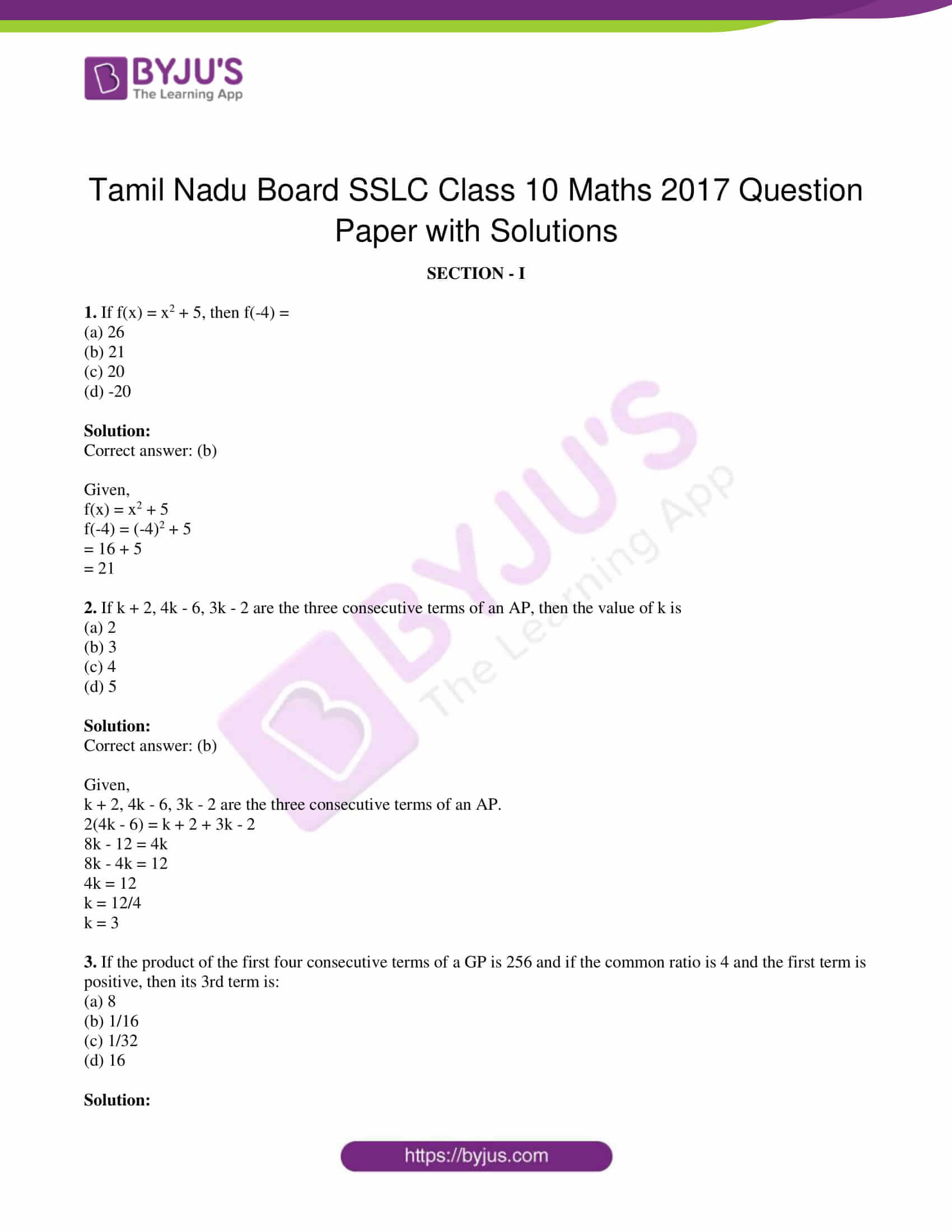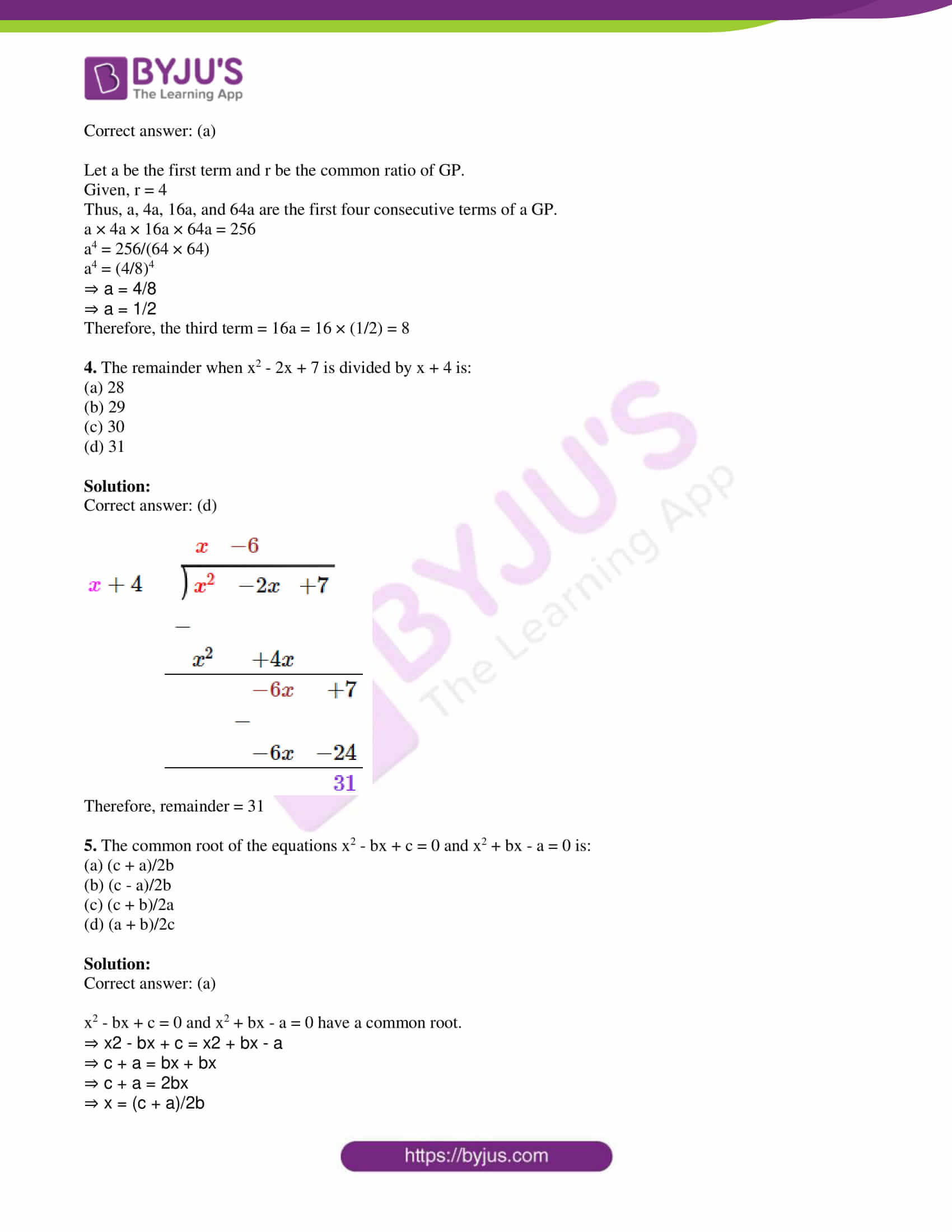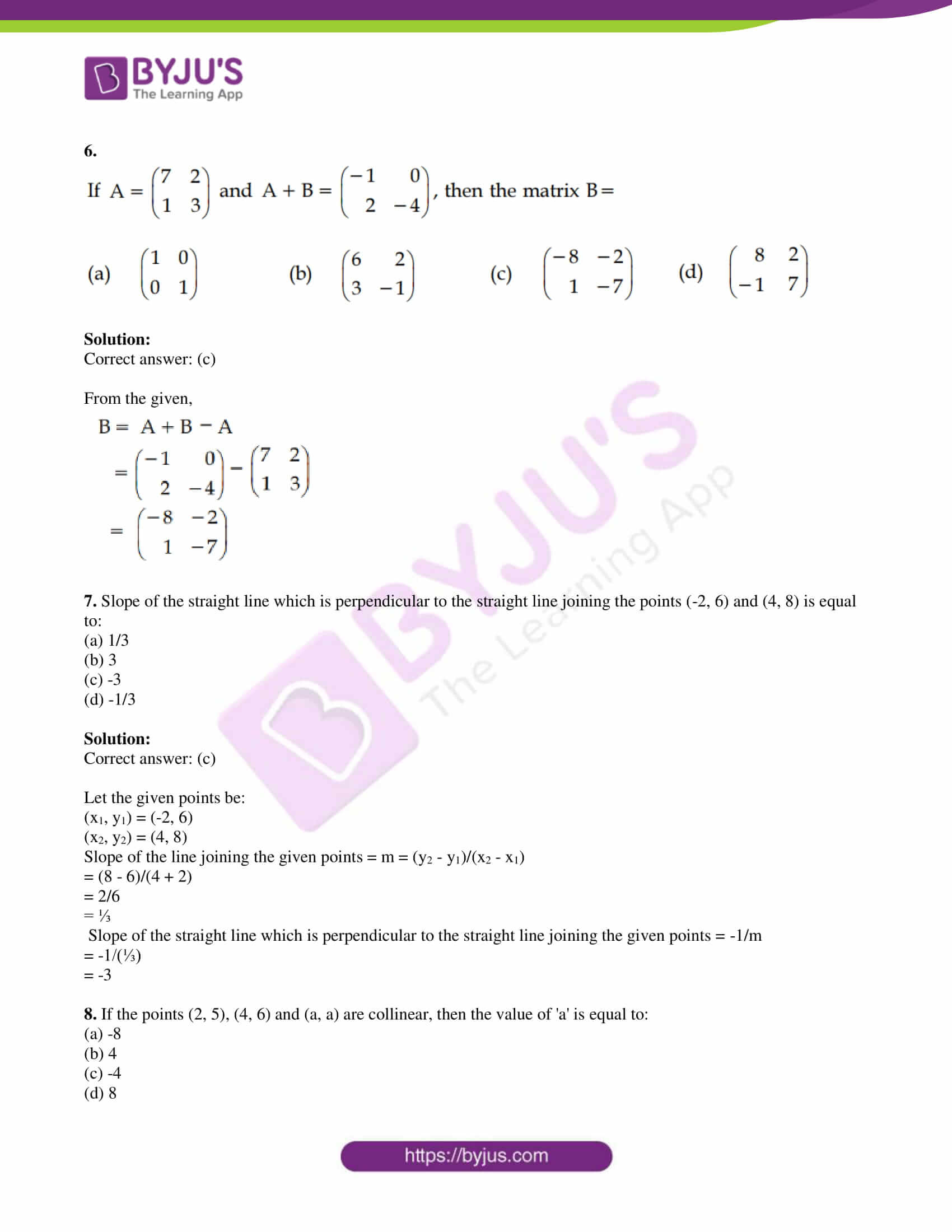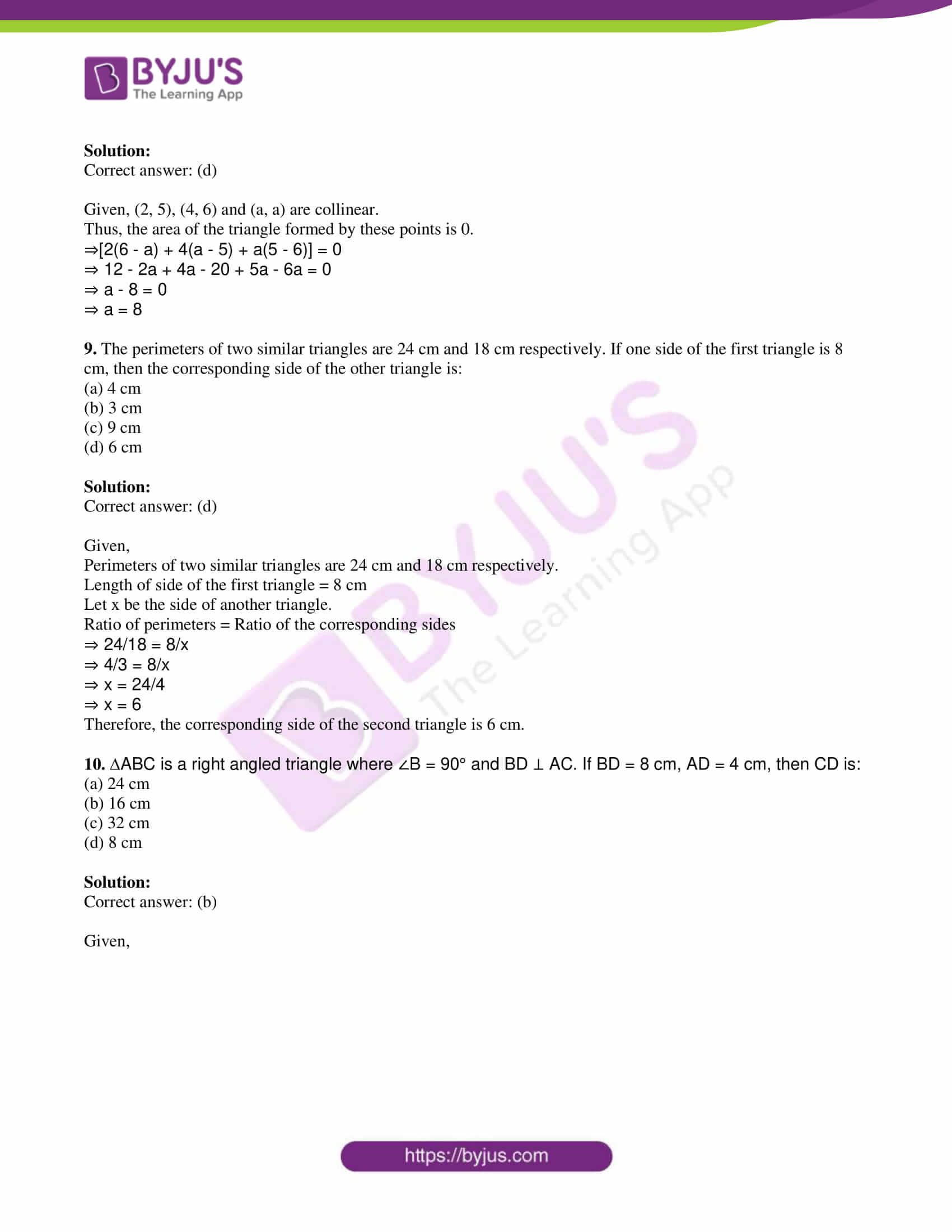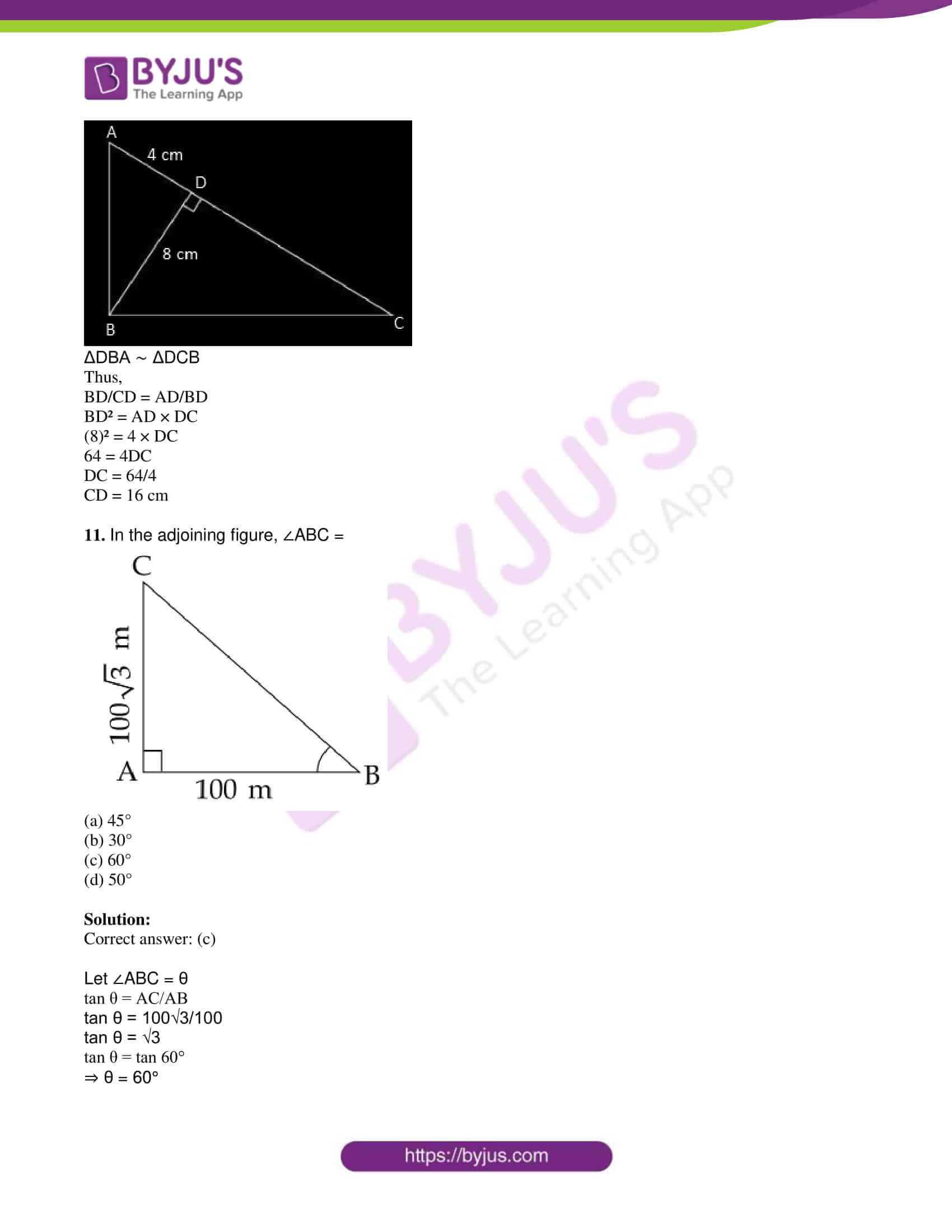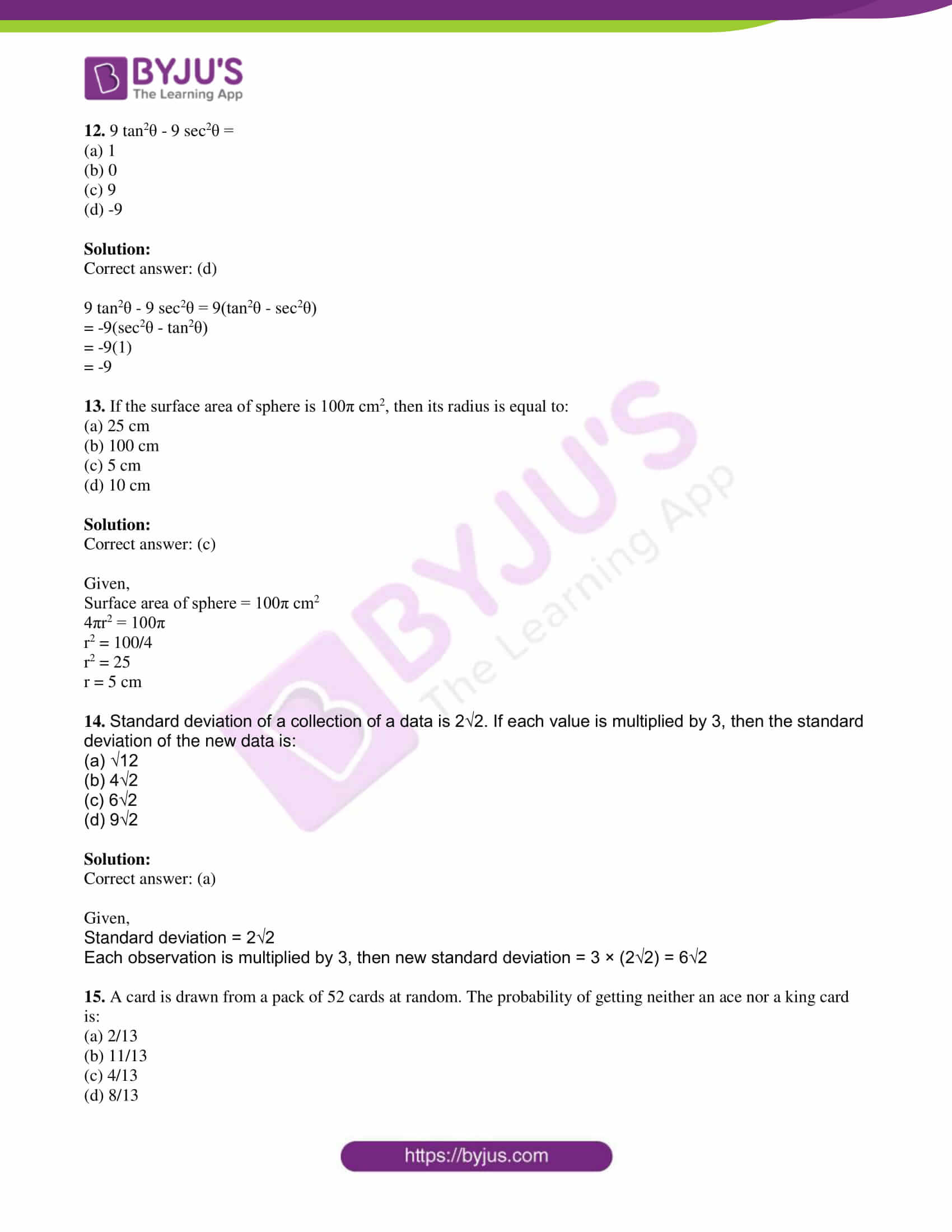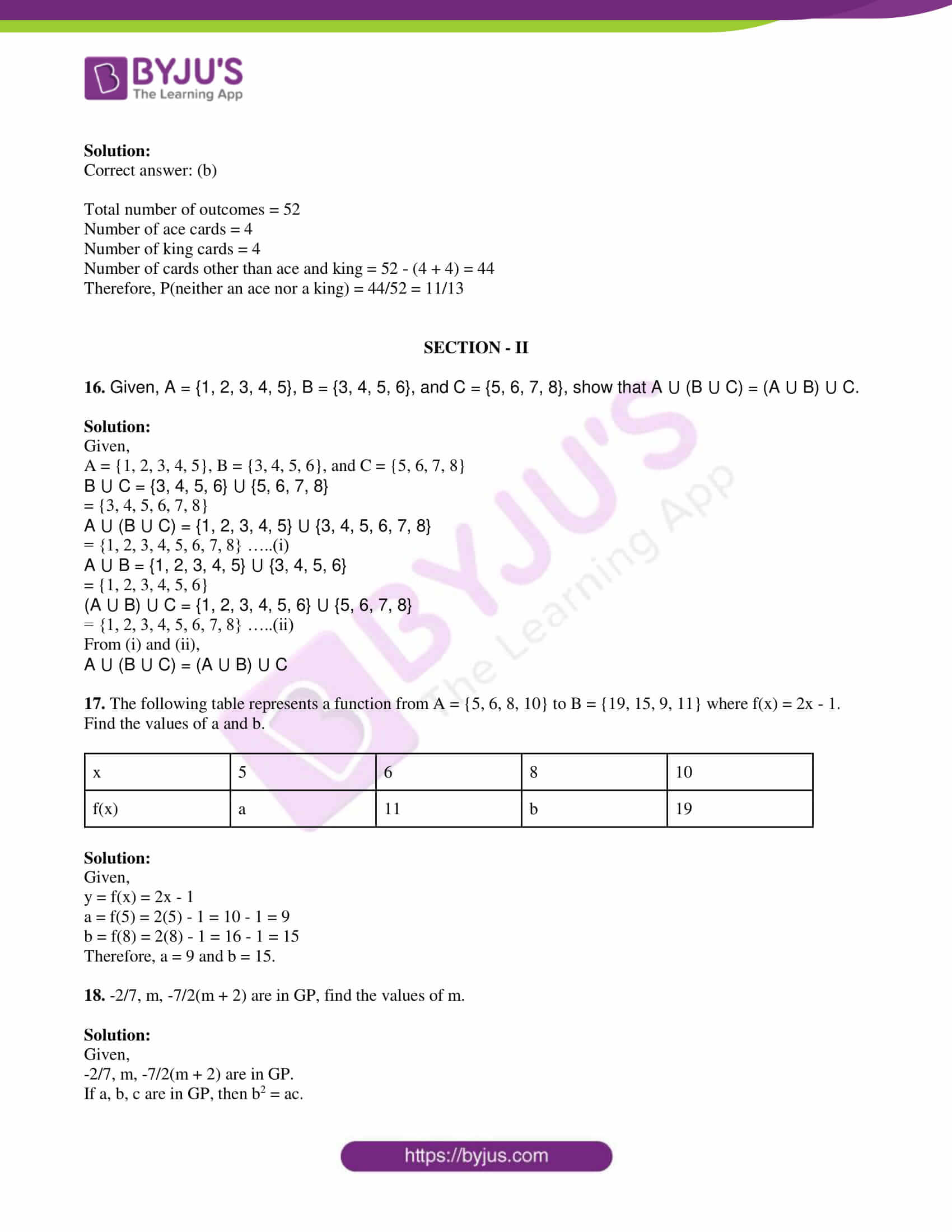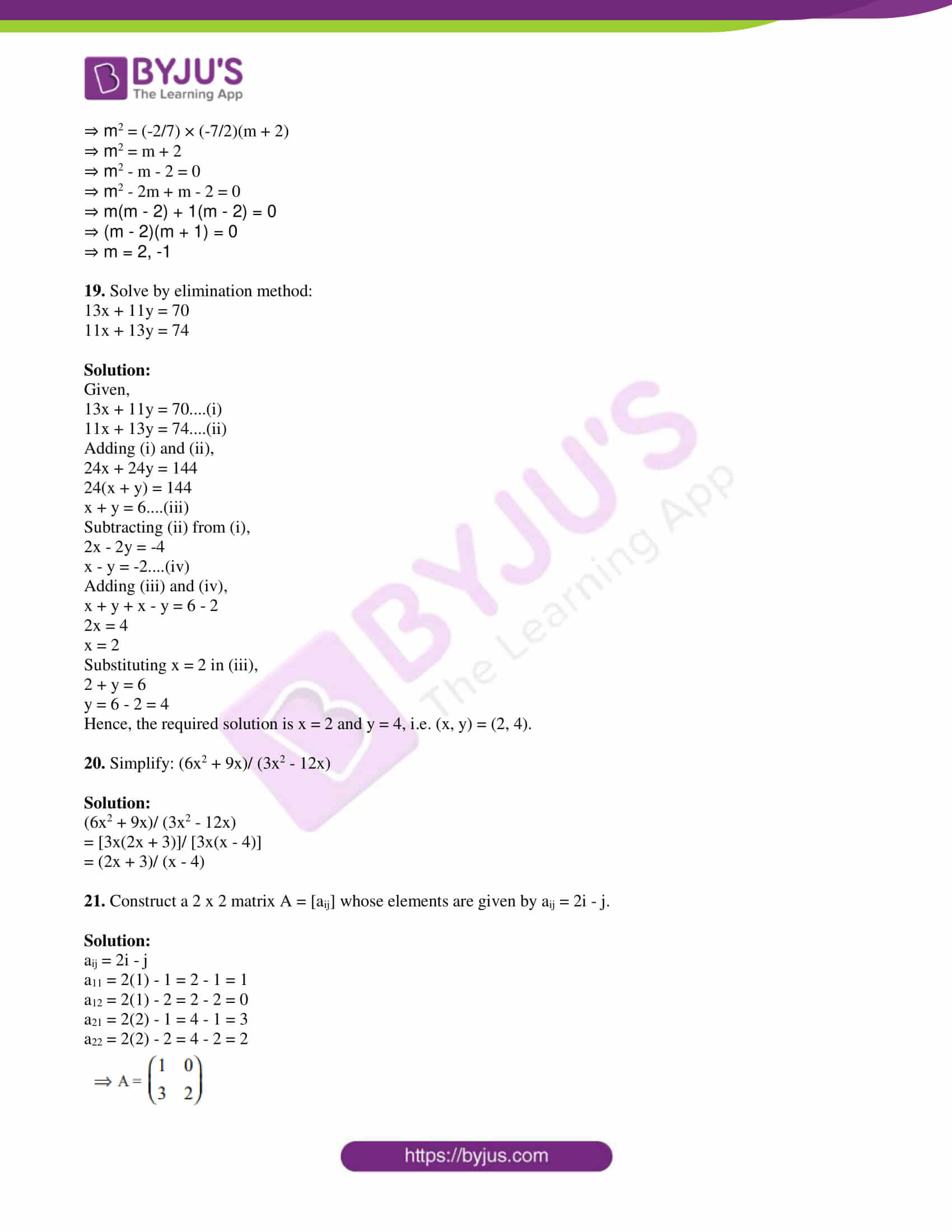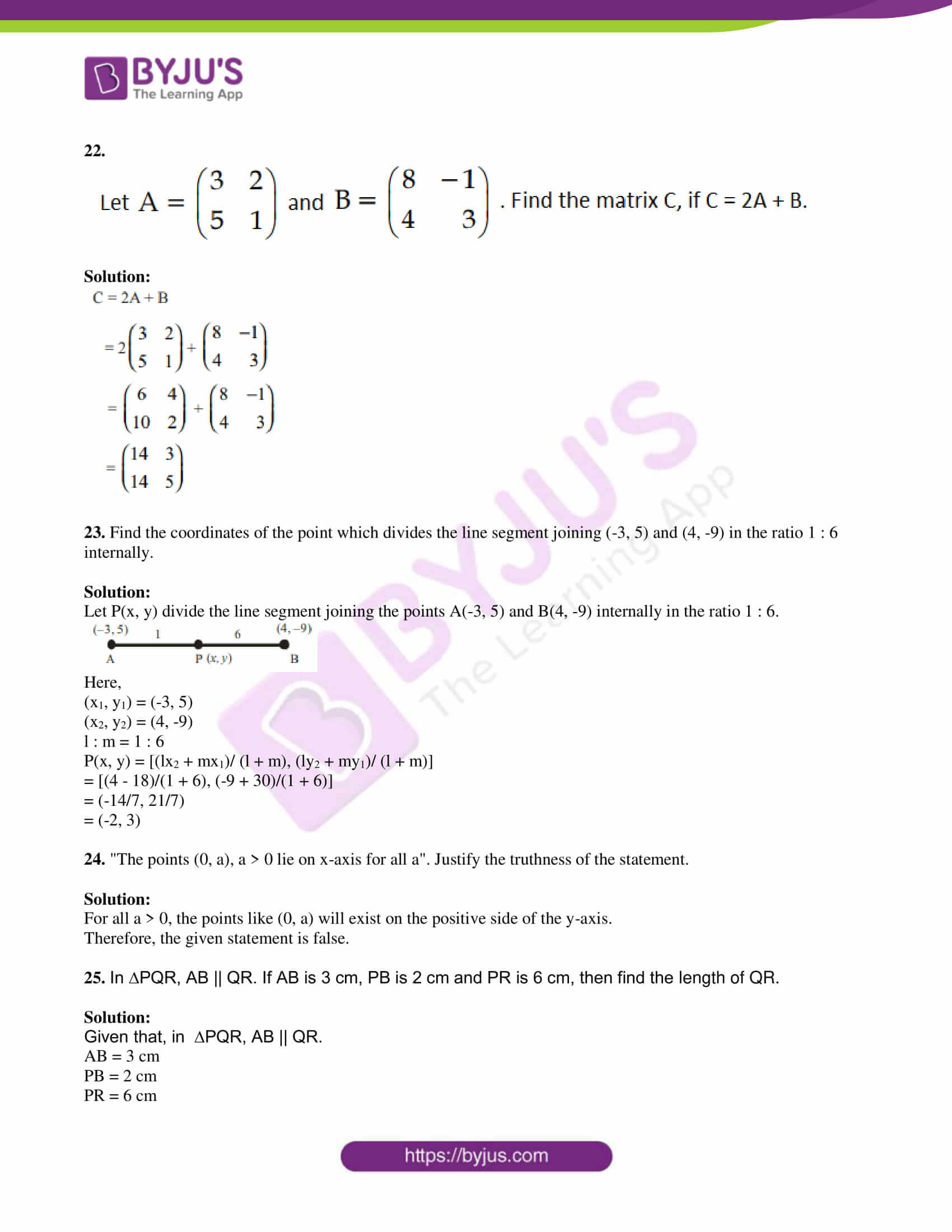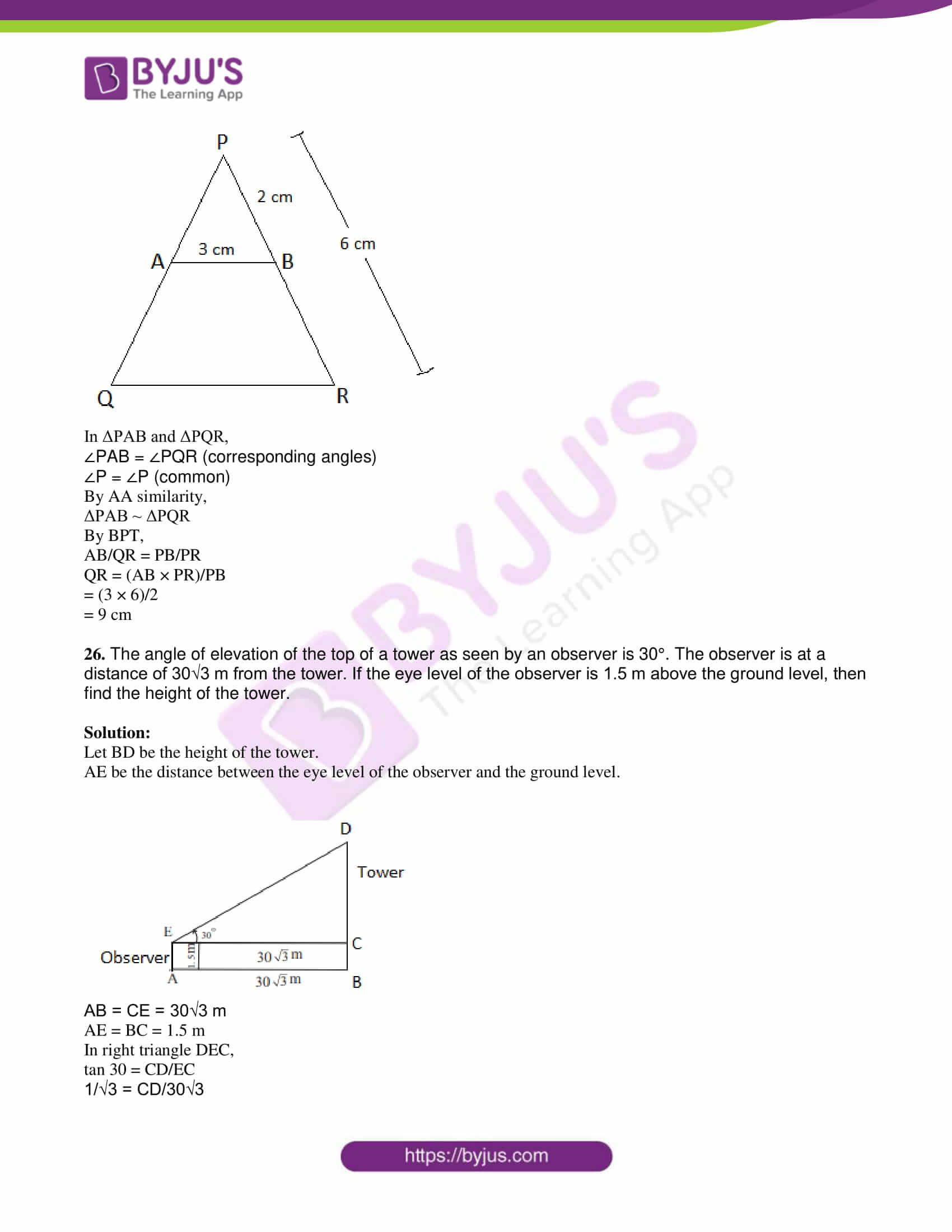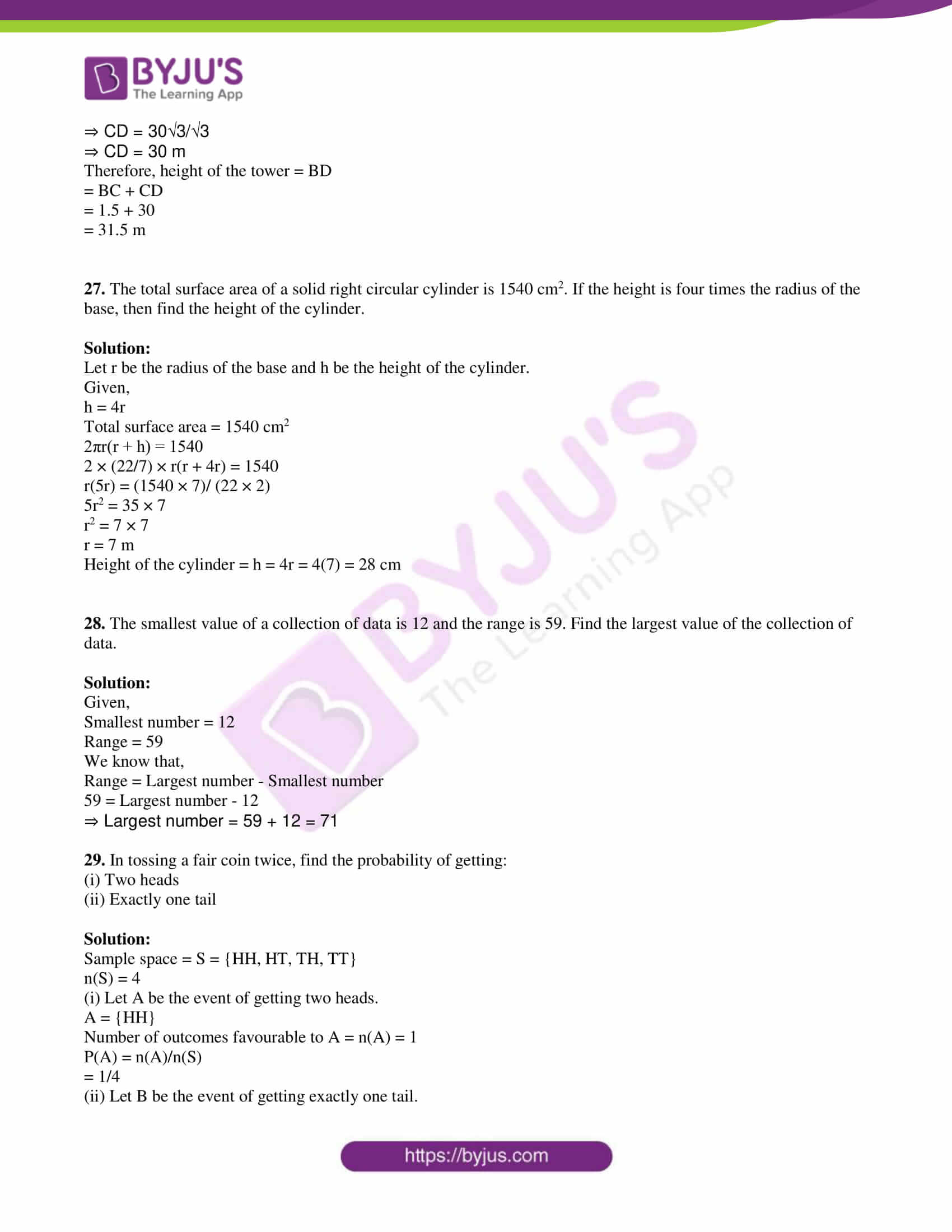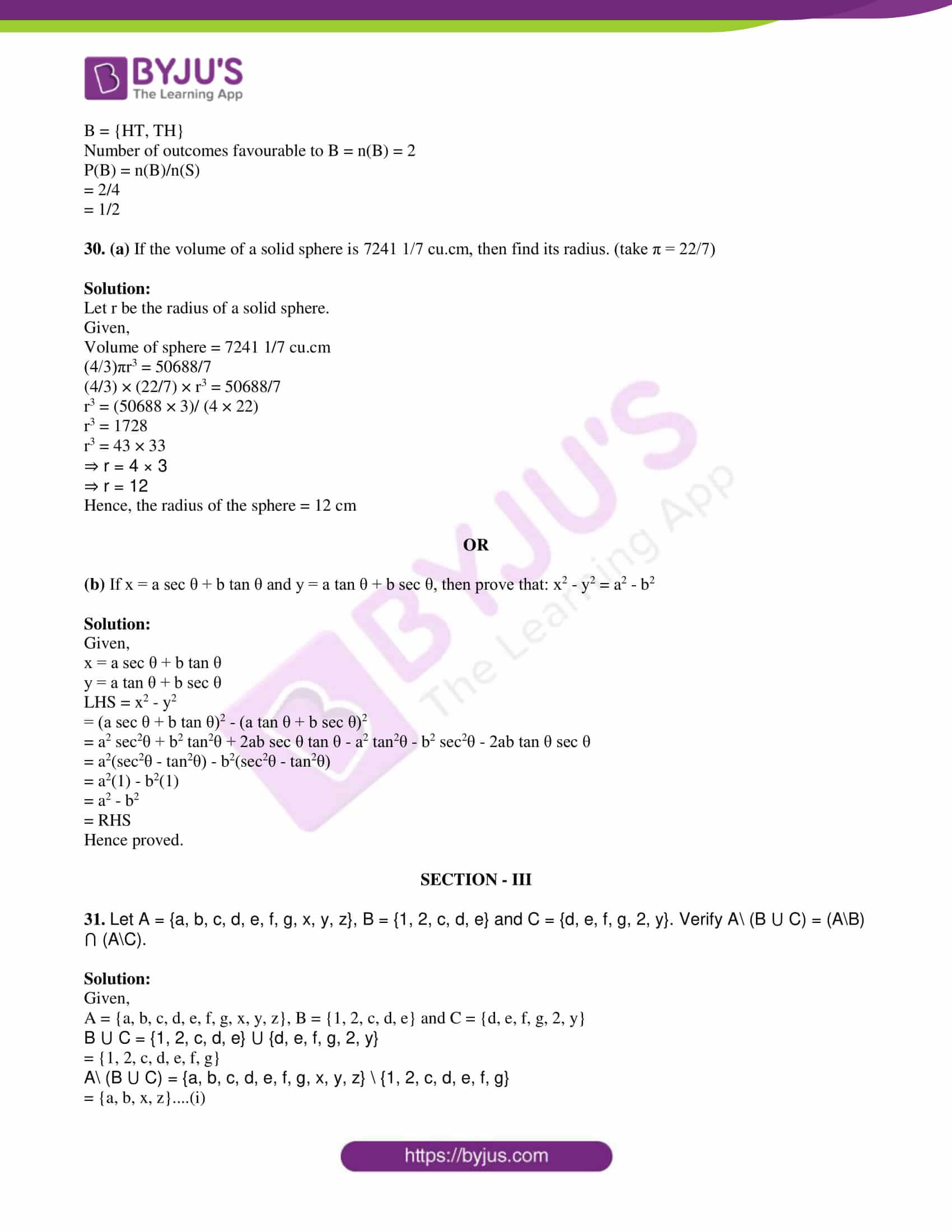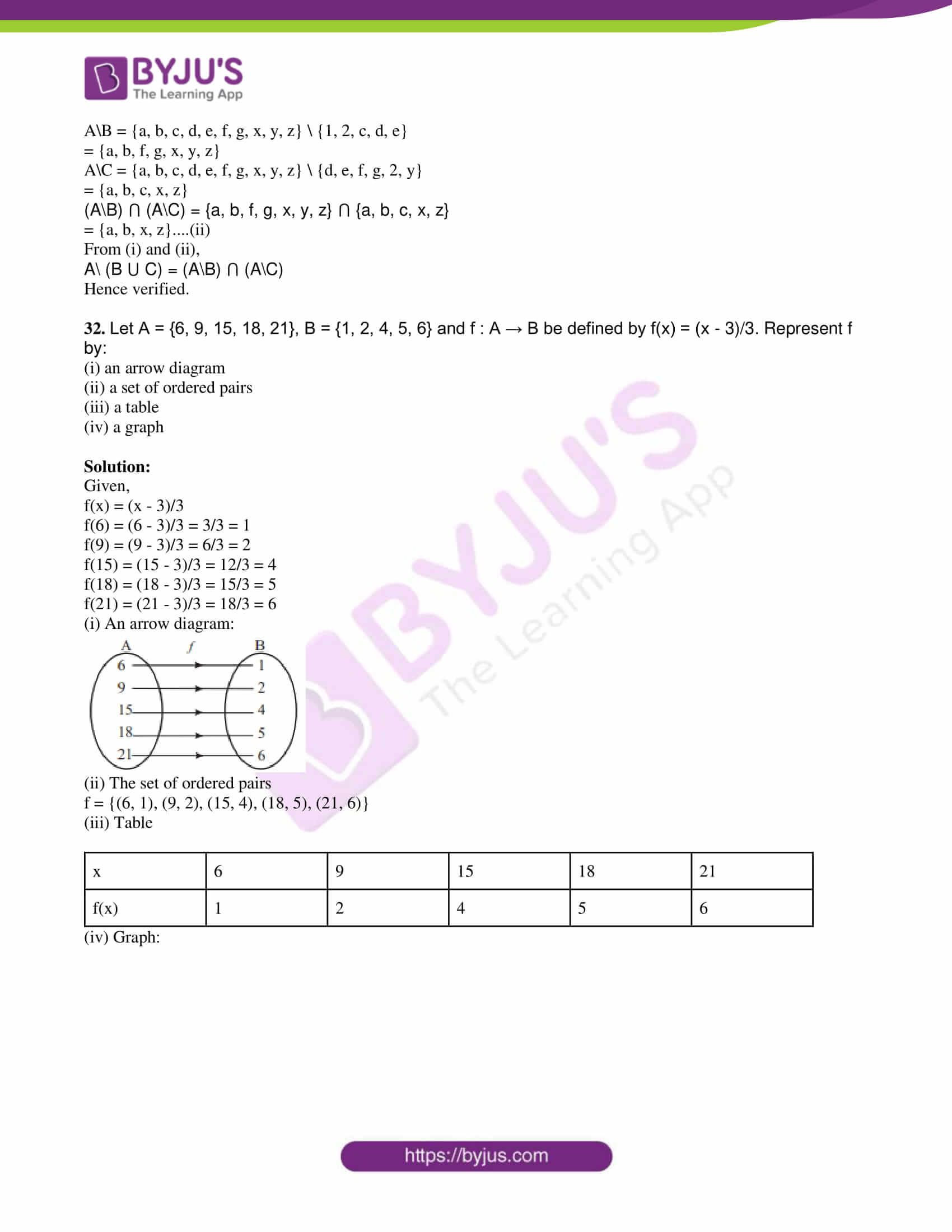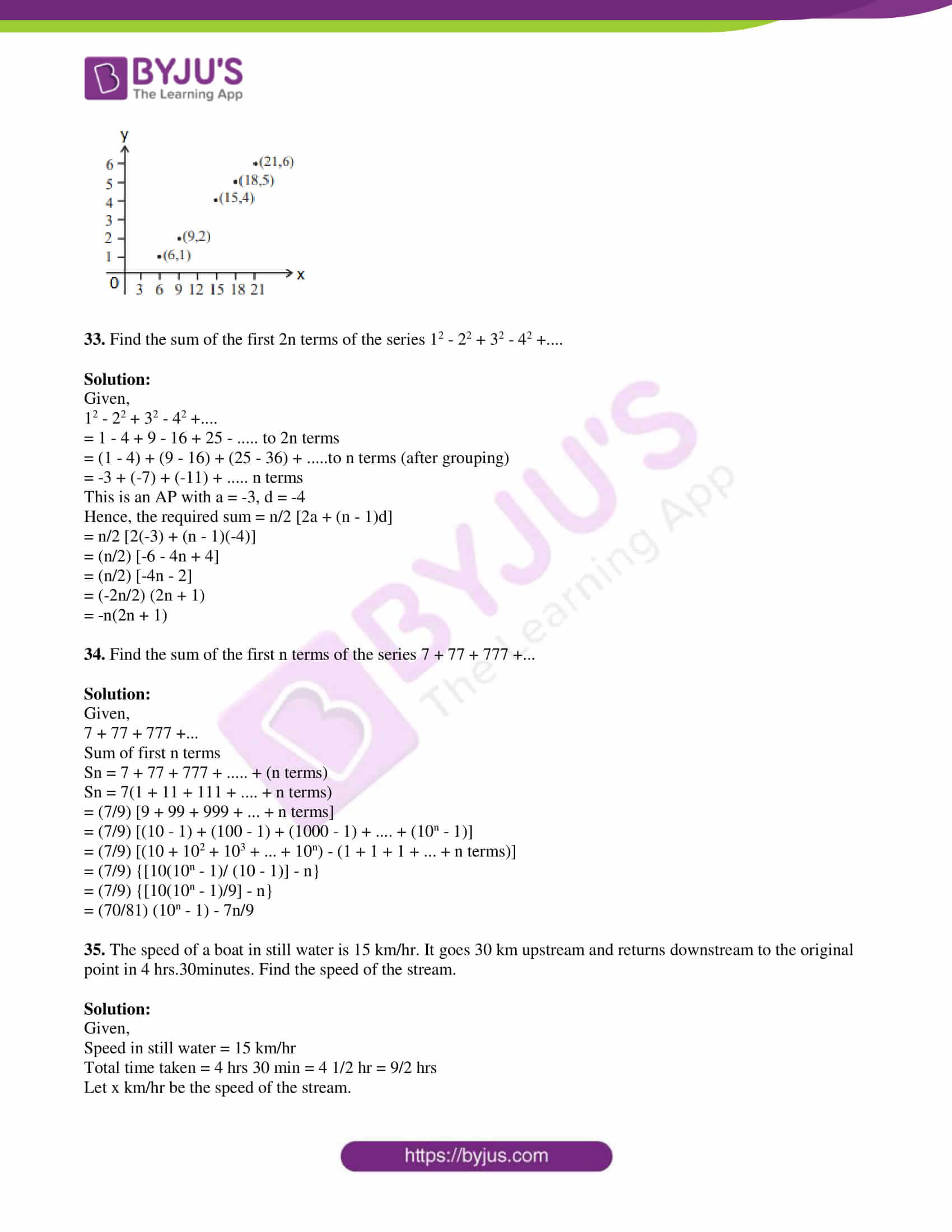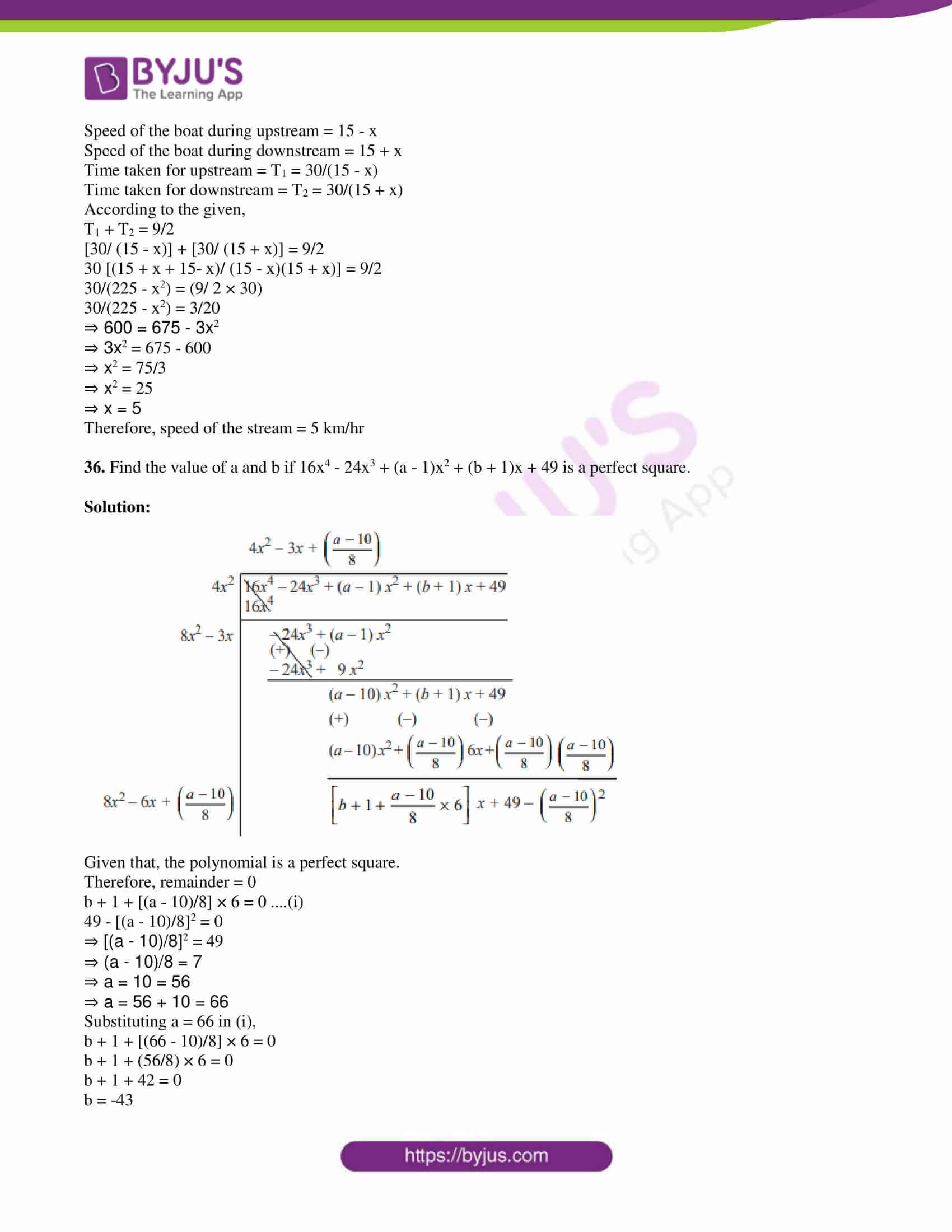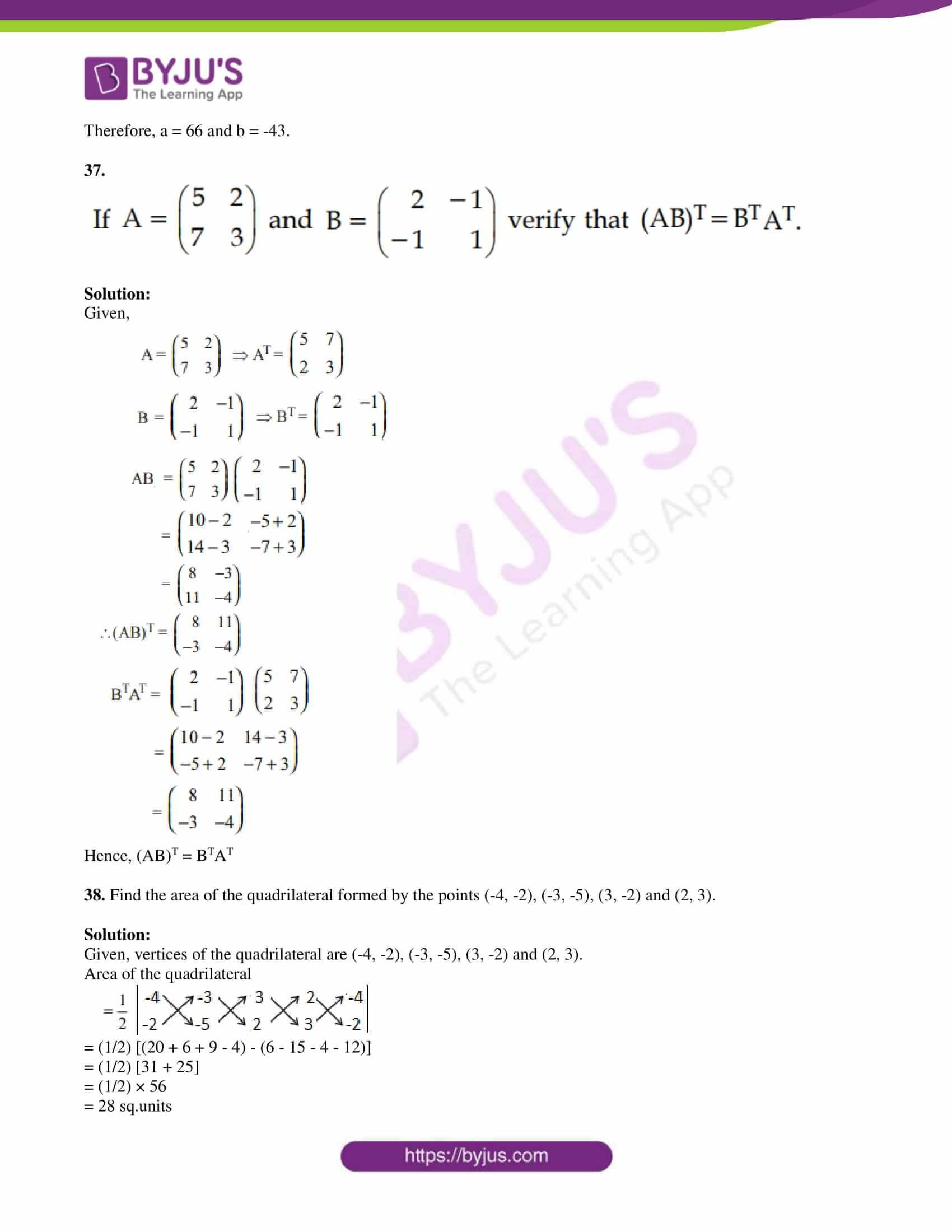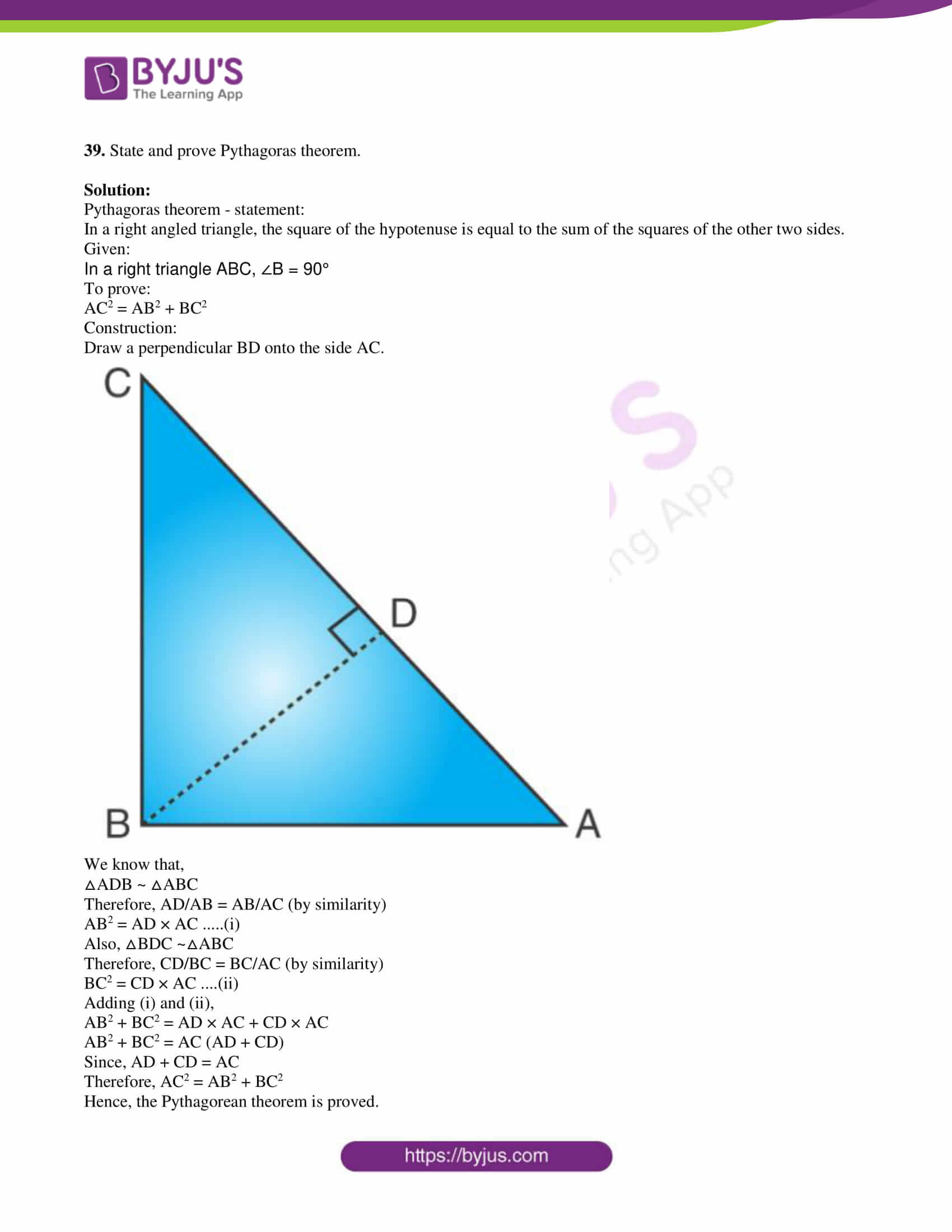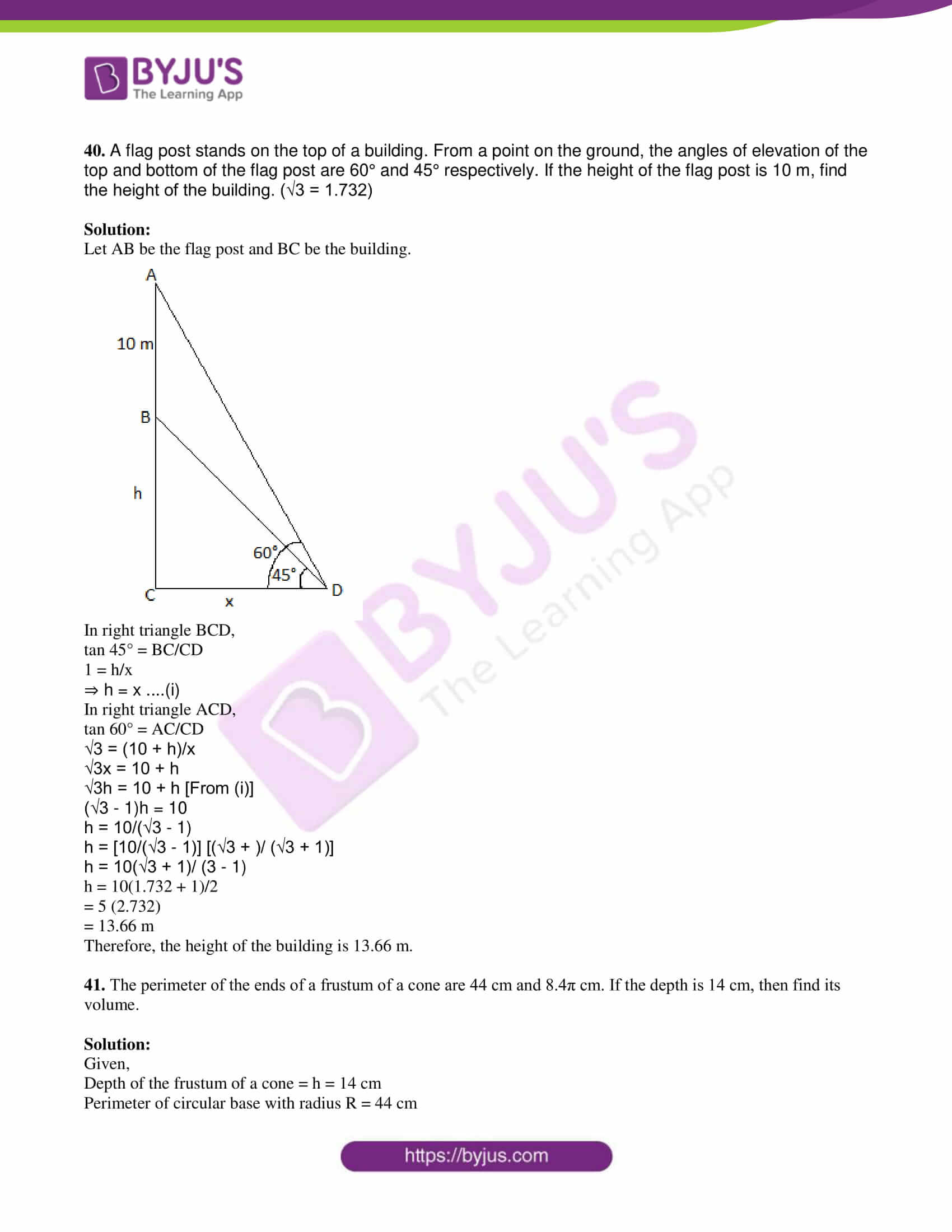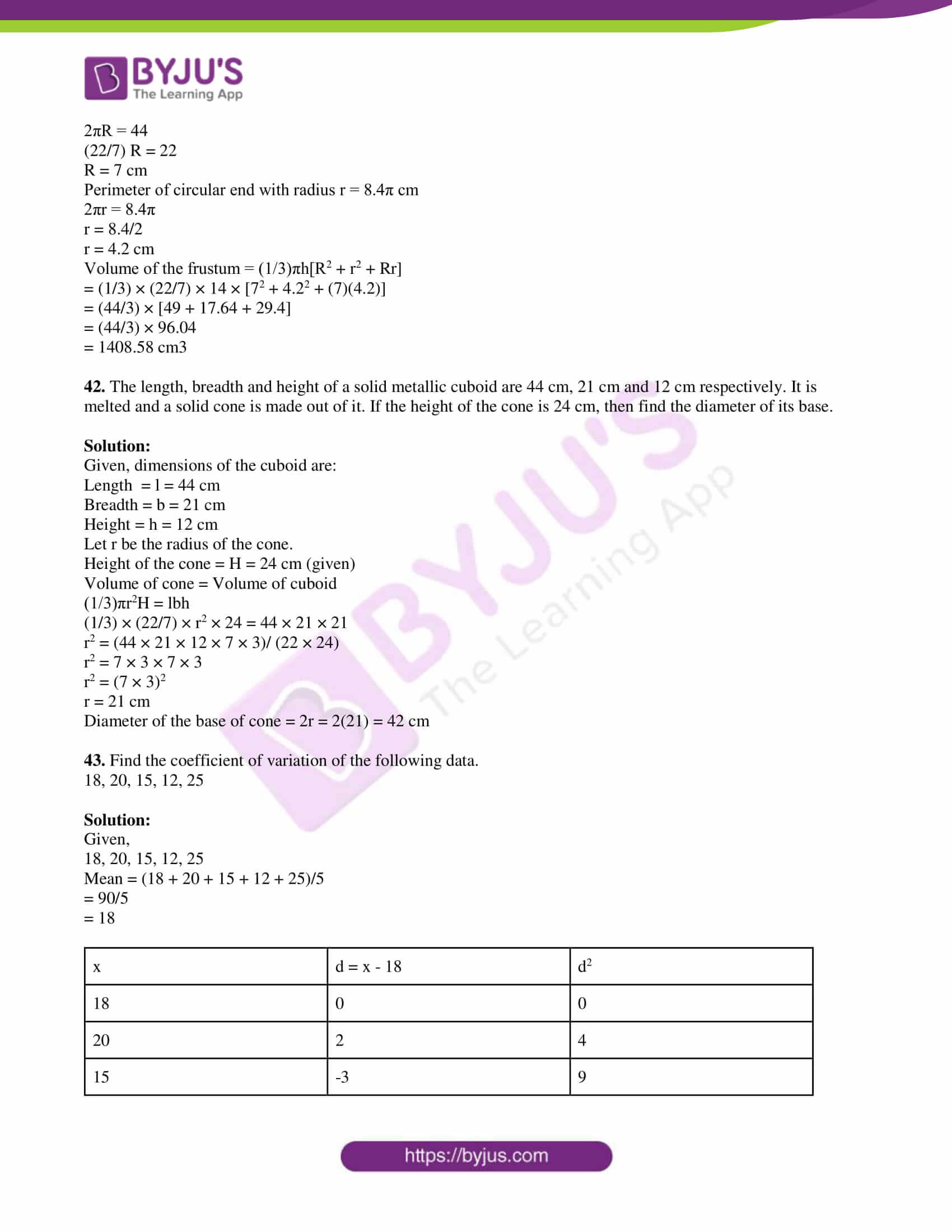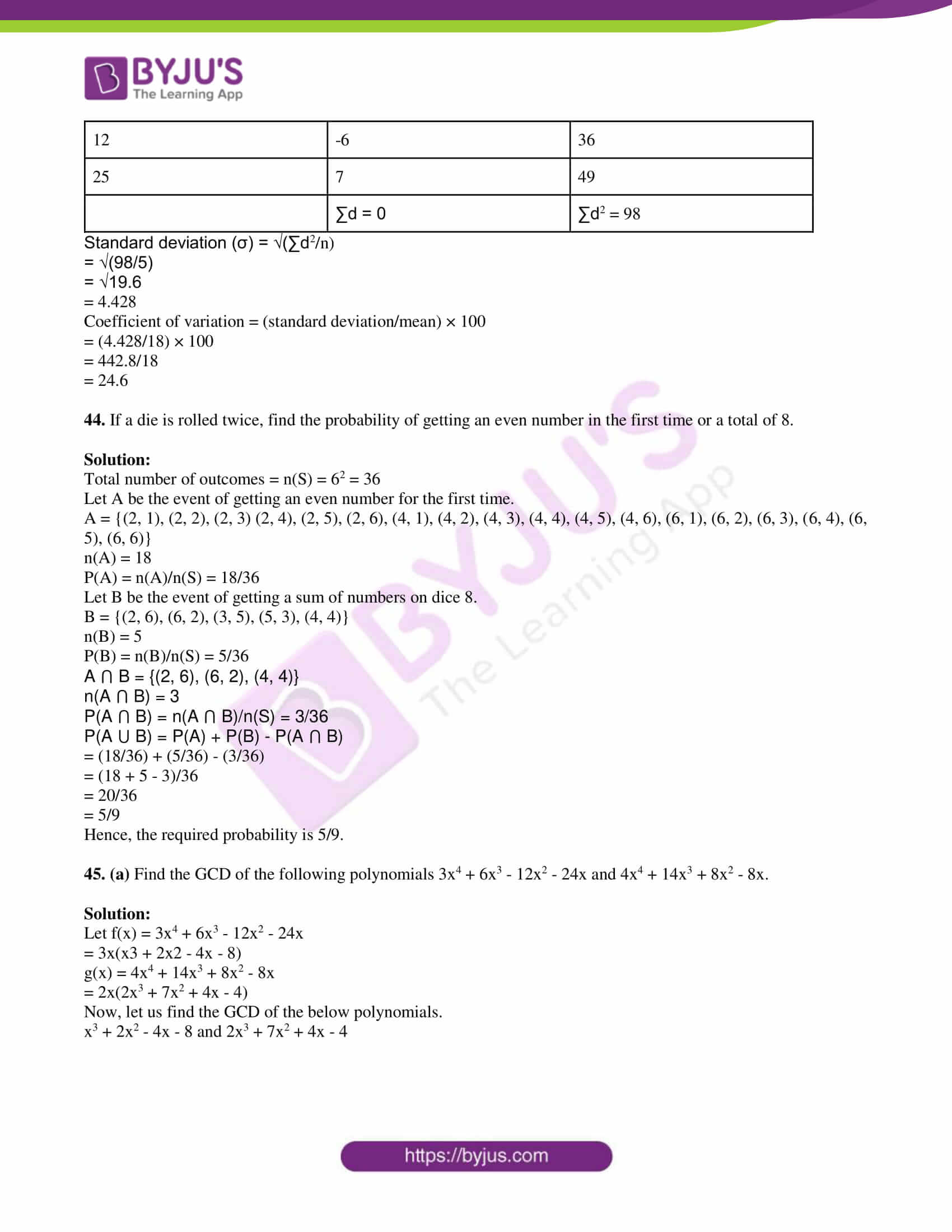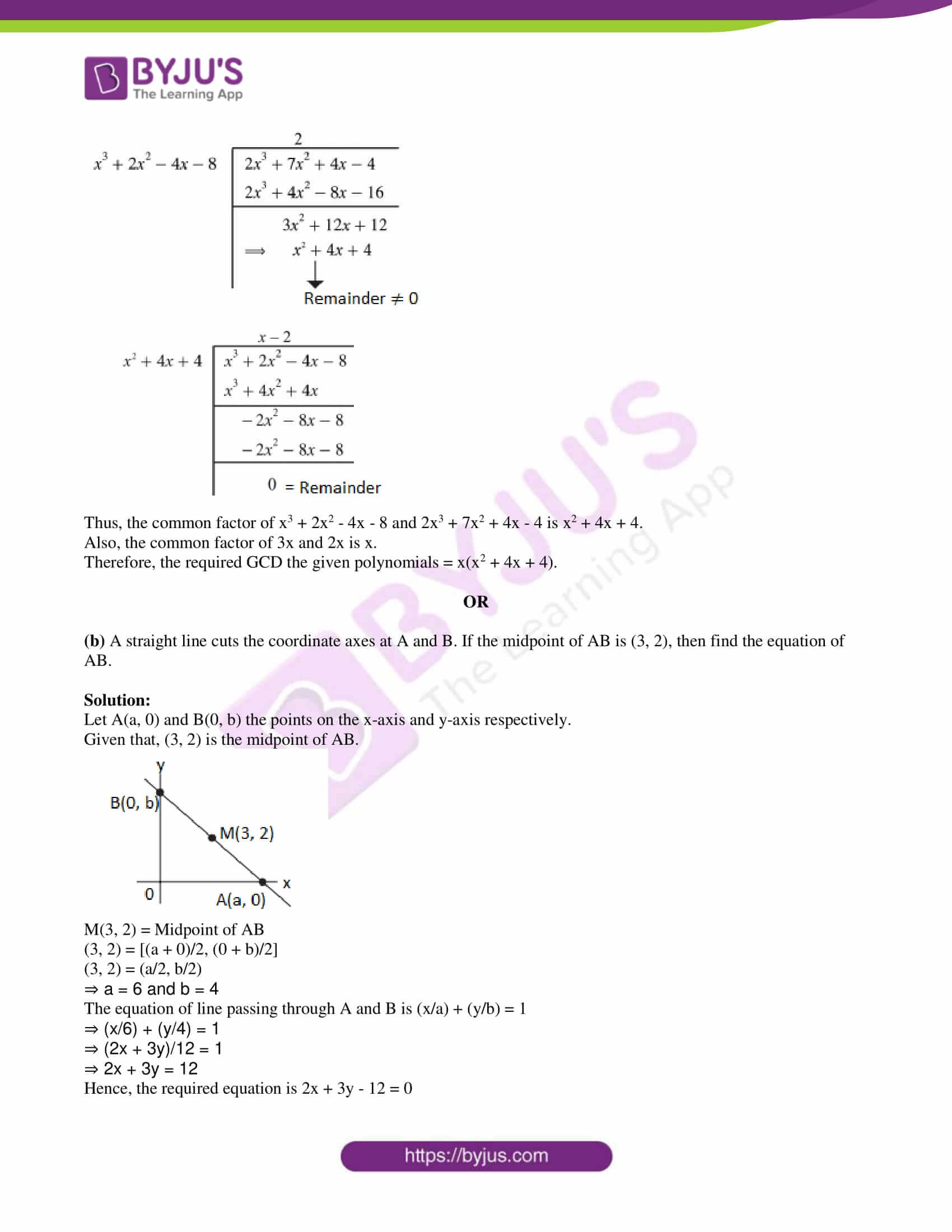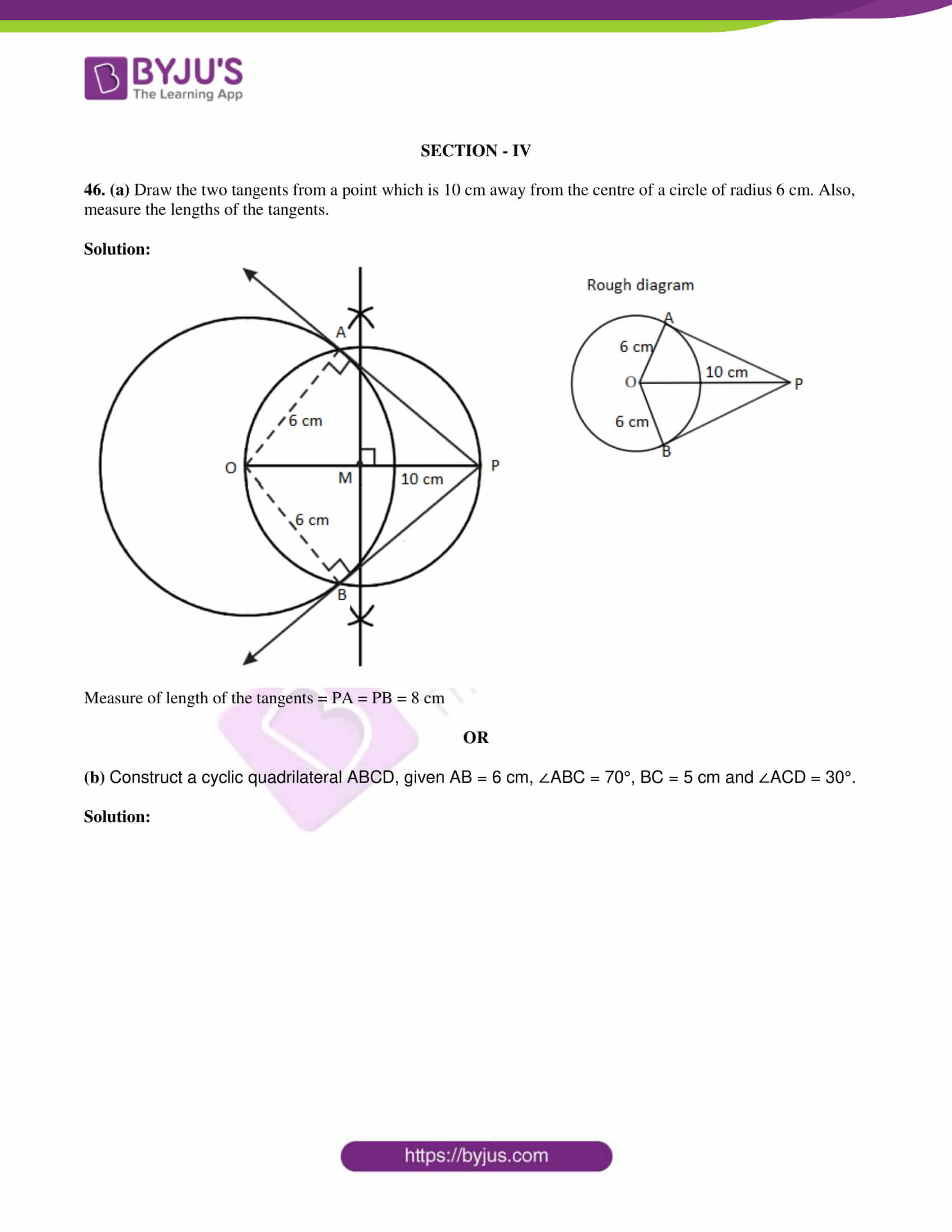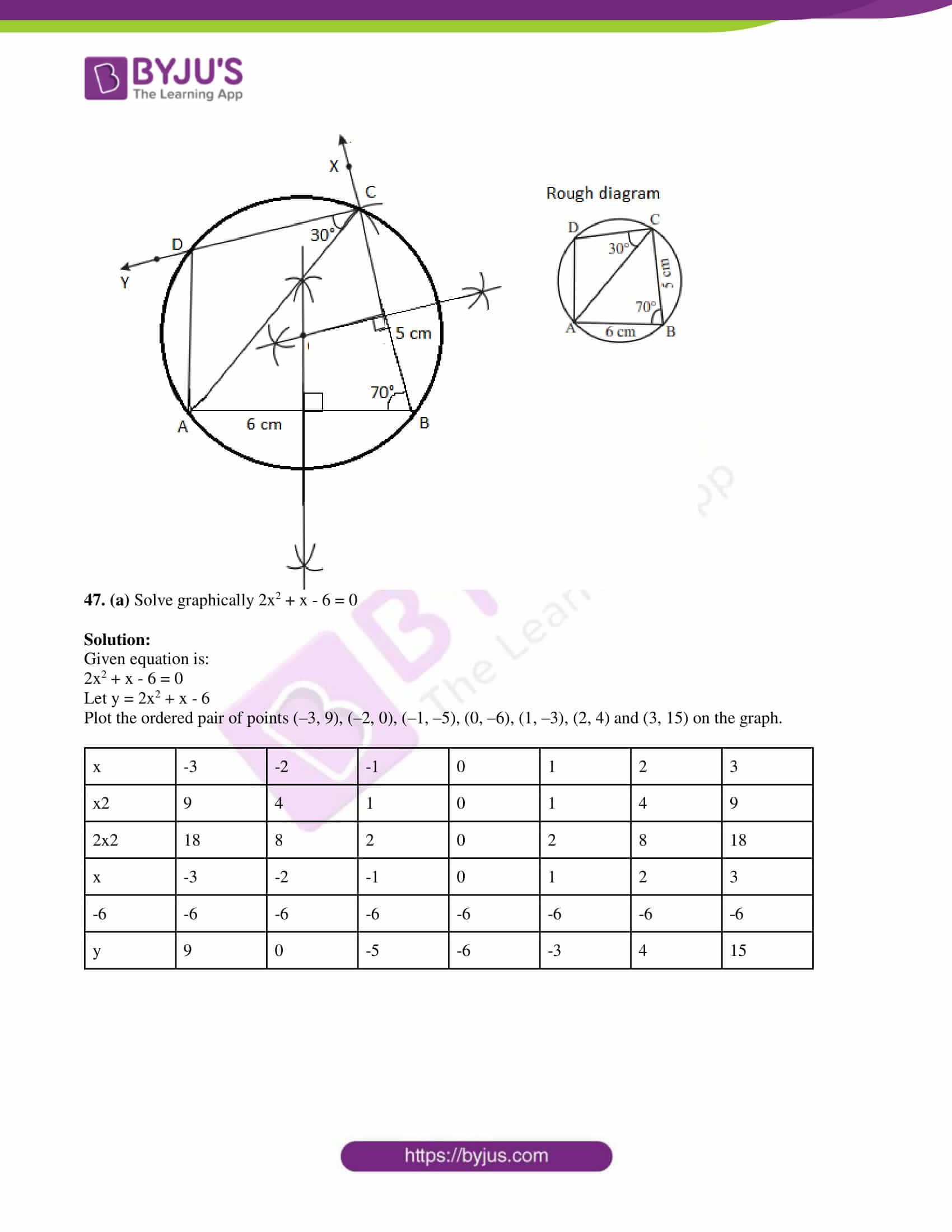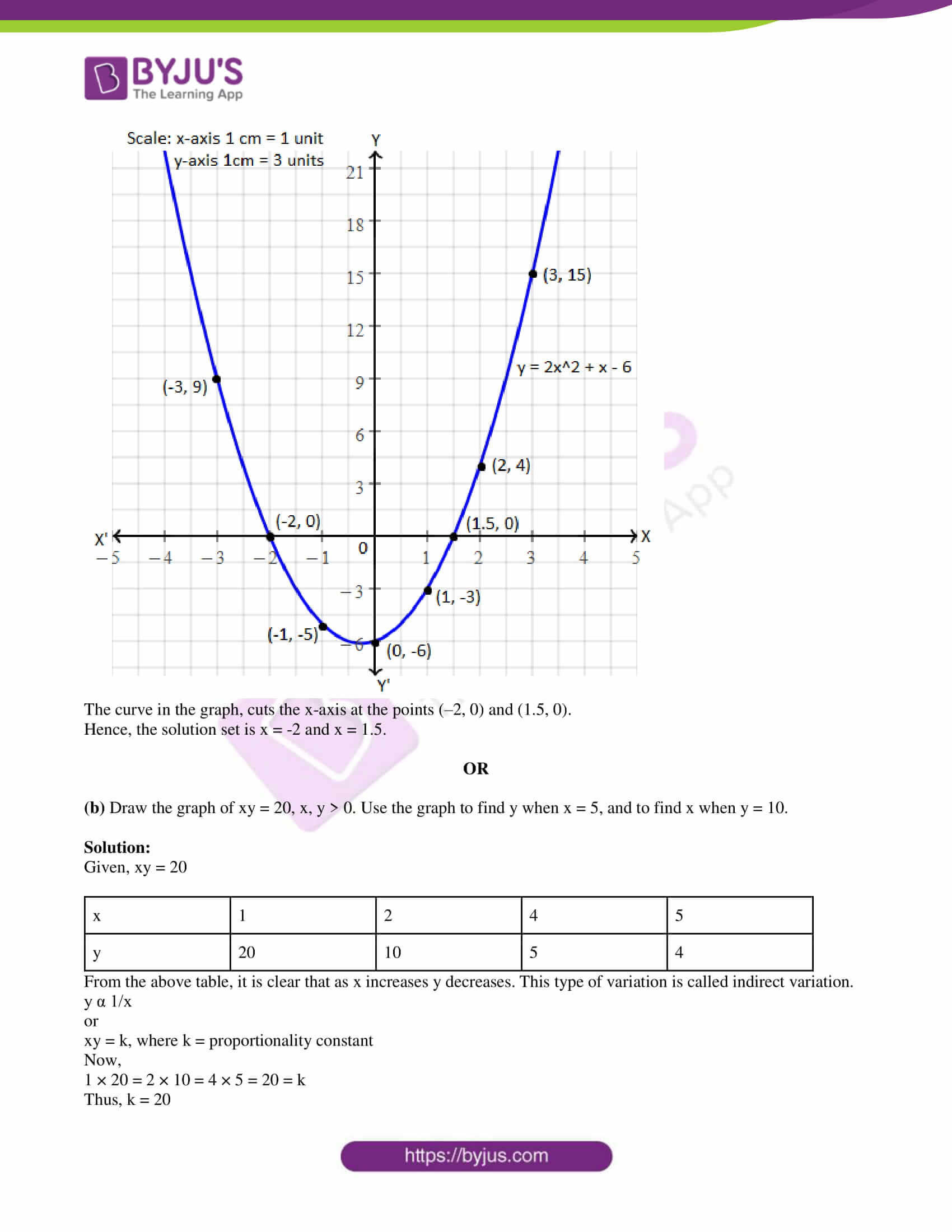SECTION – I

1. If f(x) = x2 + 5, then f(-4) =

(a) 26

(b) 21

(c) 20

(d) -20

Solution:

Given,

f(x) = x2 + 5

f(-4) = (-4)2 + 5

= 16 + 5

= 21

2. If k + 2, 4k – 6, 3k – 2 are the three consecutive terms of an AP, then the value of k is

(a) 2

(b) 3

(c) 4

(d) 5

Solution:

Given,

k + 2, 4k – 6, 3k – 2 are the three consecutive terms of an AP.

2(4k – 6) = k + 2 + 3k – 2

8k – 12 = 4k

8k – 4k = 12

4k = 12

k = 12/4

k = 3

3. If the product of the first four consecutive terms of a GP is 256 and if the common ratio is 4 and the first term is positive, then its 3rd term is:

(a) 8

(b) 1/16

(c) 1/32

(d) 16

Solution:

Let a be the first term and r be the common ratio of GP.

Given, r = 4

Thus, a, 4a, 16a, and 64a are the first four consecutive terms of a GP.

a × 4a × 16a × 64a = 256

a4 = 256/(64 × 64)

a4 = (4/8)4

⇒ a = 4/8

⇒ a = 1/2

Therefore, the third term = 16a = 16 × (1/2) = 8

4. The remainder when x2 – 2x + 7 is divided by x + 4 is:

(a) 28

(b) 29

(c) 30

(d) 31

Solution: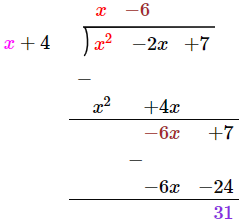Therefore, remainder = 31

5. The common root of the equations x2 – bx + c = 0 and x2 + bx – a = 0 is:

(a) (c + a)/2b

(b) (c – a)/2b

(c) (c + b)/2a

(d) (a + b)/2c

Solution:

x2 – bx + c = 0 and x2 + bx – a = 0 have a common root.

⇒ x2 – bx + c = x2 + bx – a

⇒ c + a = bx + bx

⇒ c + a = 2bx

⇒ x = (c + a)/2b

6.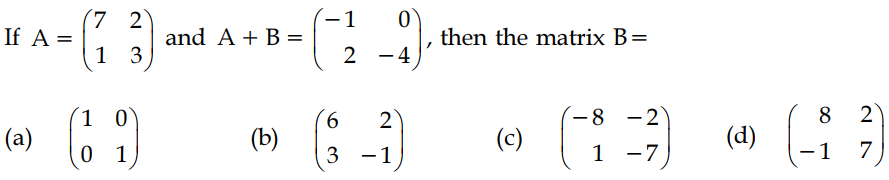Solution:

From the given,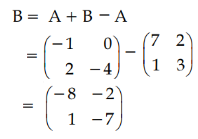7. Slope of the straight line which is perpendicular to the straight line joining the points (-2, 6) and (4, 8) is equal to:

(a) 1/3

(b) 3

(c) -3

(d) -1/3

Solution:

Let the given points be:

(x1, y1) = (-2, 6)

(x2, y2) = (4, 8)

Slope of the line joining the given points = m = (y2 – y1)/(x2 – x1)

= (8 – 6)/(4 + 2)

= 2/6

= ⅓

Slope of the straight line which is perpendicular to the straight line joining the given points = -1/m

= -1/(⅓)

= -3

8. If the points (2, 5), (4, 6) and (a, a) are collinear, then the value of ‘a’ is equal to:

(a) -8

(b) 4

(c) -4

(d) 8

Solution:

Given, (2, 5), (4, 6) and (a, a) are collinear.

Thus, the area of the triangle formed by these points is 0.

⇒[2(6 – a) + 4(a – 5) + a(5 – 6)] = 0

⇒ 12 – 2a + 4a – 20 + 5a – 6a = 0

⇒ a – 8 = 0

⇒ a = 8

9. The perimeters of two similar triangles are 24 cm and 18 cm respectively. If one side of the first triangle is 8 cm, then the corresponding side of the other triangle is:

(a) 4 cm

(b) 3 cm

(c) 9 cm

(d) 6 cm

Solution:

Given,

Perimeters of two similar triangles are 24 cm and 18 cm respectively.

Length of side of the first triangle = 8 cm

Let x be the side of another triangle.

Ratio of perimeters = Ratio of the corresponding sides

⇒ 24/18 = 8/x

⇒ 4/3 = 8/x

⇒ x = 24/4

⇒ x = 6

Therefore, the corresponding side of the second triangle is 6 cm.

10. ∆ABC is a right angled triangle where ∠B = 90° and BD ⊥ AC. If BD = 8 cm, AD = 4 cm, then CD is:

(a) 24 cm

(b) 16 cm

(c) 32 cm

(d) 8 cm

Solution:

Given,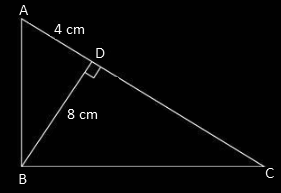ΔDBA ∼ ΔDCB

Thus,

(8)² = 4 × DC

64 = 4DC

DC = 64/4

CD = 16 cm

11. In the adjoining figure, ∠ABC =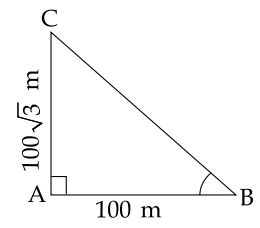(a) 45°

(b) 30°

(c) 60°

(d) 50°

Solution:

Let ∠ABC = θ

tan θ = AC/AB

tan θ = 100√3/100

tan θ = √3

tan θ = tan 60°

⇒ θ = 60°

12. 9 tan2θ – 9 sec2θ =

(a) 1

(b) 0

(c) 9

(d) -9

Solution:

9 tan2θ – 9 sec2θ = 9(tan2θ – sec2θ)

= -9(sec2θ – tan2θ)

= -9(1)

= -9

13. If the surface area of sphere is 100π cm2, then its radius is equal to:

(a) 25 cm

(b) 100 cm

(c) 5 cm

(d) 10 cm

Solution:

Given,

Surface area of sphere = 100π cm2

4πr2 = 100π

r2 = 100/4

r2 = 25

r = 5 cm

14. Standard deviation of a collection of a data is 2√2. If each value is multiplied by 3, then the standard deviation of the new data is:

(a) √12

(b) 4√2

(c) 6√2

(d) 9√2

Solution:

Given,

Standard deviation = 2√2

Each observation is multiplied by 3, then new standard deviation = 3 × (2√2) = 6√2

15. A card is drawn from a pack of 52 cards at random. The probability of getting neither an ace nor a king card is:

(a) 2/13

(b) 11/13

(c) 4/13

(d) 8/13

Solution:

Total number of outcomes = 52

Number of ace cards = 4

Number of king cards = 4

Number of cards other than ace and king = 52 – (4 + 4) = 44

Therefore, P(neither an ace nor a king) = 44/52 = 11/13

SECTION – II

16. Given, A = {1, 2, 3, 4, 5}, B = {3, 4, 5, 6}, and C = {5, 6, 7, 8}, show that A ⋃ (B ⋃ C) = (A ⋃ B) ⋃ C.

Solution:

Given,

A = {1, 2, 3, 4, 5}, B = {3, 4, 5, 6}, and C = {5, 6, 7, 8}

B ⋃ C = {3, 4, 5, 6} ⋃ {5, 6, 7, 8}

= {3, 4, 5, 6, 7, 8}

A ⋃ (B ⋃ C) = {1, 2, 3, 4, 5} ⋃ {3, 4, 5, 6, 7, 8}

= {1, 2, 3, 4, 5, 6, 7, 8} …..(i)

A ⋃ B = {1, 2, 3, 4, 5} ⋃ {3, 4, 5, 6}

= {1, 2, 3, 4, 5, 6}

(A ⋃ B) ⋃ C = {1, 2, 3, 4, 5, 6} ⋃ {5, 6, 7, 8}

= {1, 2, 3, 4, 5, 6, 7, 8} …..(ii)

From (i) and (ii),

A ⋃ (B ⋃ C) = (A ⋃ B) ⋃ C

17. The following table represents a function from A = {5, 6, 8, 10} to B = {19, 15, 9, 11} where f(x) = 2x – 1. Find the values of a and b.

 x 5 6 8 10 f(x) a 11 b 19

Solution:

Given,

y = f(x) = 2x – 1

a = f(5) = 2(5) – 1 = 10 – 1 = 9

b = f(8) = 2(8) – 1 = 16 – 1 = 15

Therefore, a = 9 and b = 15.

18. -2/7, m, -7/2(m + 2) are in GP, find the values of m.

Solution:

Given,

-2/7, m, -7/2(m + 2) are in GP.

If a, b, c are in GP, then b2 = ac.

⇒ m2 = (-2/7) × (-7/2)(m + 2)

⇒ m2 = m + 2

⇒ m2 – m – 2 = 0

⇒ m2 – 2m + m – 2 = 0

⇒ m(m – 2) + 1(m – 2) = 0

⇒ (m – 2)(m + 1) = 0

⇒ m = 2, -1

19. Solve by elimination method:

13x + 11y = 70

11x + 13y = 74

Solution:

Given,

13x + 11y = 70….(i)

11x + 13y = 74….(ii)

24x + 24y = 144

24(x + y) = 144

x + y = 6….(iii)

Subtracting (ii) from (i),

2x – 2y = -4

x – y = -2….(iv)

x + y + x – y = 6 – 2

2x = 4

x = 2

Substituting x = 2 in (iii),

2 + y = 6

y = 6 – 2 = 4

Hence, the required solution is x = 2 and y = 4, i.e. (x, y) = (2, 4).

20. Simplify: (6x2 + 9x)/ (3x2 – 12x)

Solution:

(6x2 + 9x)/ (3x2 – 12x)

= [3x(2x + 3)]/ [3x(x – 4)]

= (2x + 3)/ (x – 4)

21. Construct a 2 x 2 matrix A = [aij] whose elements are given by aij = 2i – j.

Solution:

aij = 2i – j

a11 = 2(1) – 1 = 2 – 1 = 1

a12 = 2(1) – 2 = 2 – 2 = 0

a21 = 2(2) – 1 = 4 – 1 = 3

a22 = 2(2) – 2 = 4 – 2 = 2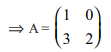22.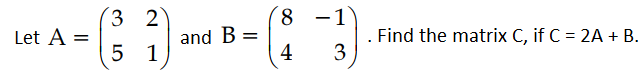Solution: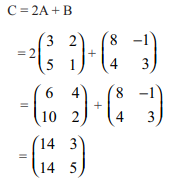23. Find the coordinates of the point which divides the line segment joining (-3, 5) and (4, -9) in the ratio 1 : 6 internally.

Solution:

Let P(x, y) divide the line segment joining the points A(-3, 5) and B(4, -9) internally in the ratio 1 : 6.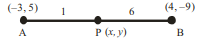Here,

(x1, y1) = (-3, 5)

(x2, y2) = (4, -9)

l : m = 1 : 6

P(x, y) = [(lx2 + mx1)/ (l + m), (ly2 + my1)/ (l + m)]

= [(4 – 18)/(1 + 6), (-9 + 30)/(1 + 6)]

= (-14/7, 21/7)

= (-2, 3)

24. “The points (0, a), a > 0 lie on x-axis for all a”. Justify the truthness of the statement.

Solution:

For all a > 0, the points like (0, a) will exist on the positive side of the y-axis.

Therefore, the given statement is false.

25. In ∆PQR, AB || QR. If AB is 3 cm, PB is 2 cm and PR is 6 cm, then find the length of QR.

Solution:

Given that, in ∆PQR, AB || QR.

AB = 3 cm

PB = 2 cm

PR = 6 cm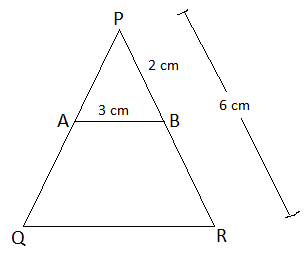In ΔPAB and ΔPQR,

∠PAB = ∠PQR (corresponding angles)

∠P = ∠P (common)

By AA similarity,

ΔPAB ~ ΔPQR

By BPT,

AB/QR = PB/PR

QR = (AB × PR)/PB

= (3 × 6)/2

= 9 cm

26. The angle of elevation of the top of a tower as seen by an observer is 30°. The observer is at a distance of 30√3 m from the tower. If the eye level of the observer is 1.5 m above the ground level, then find the height of the tower.

Solution:

Let BD be the height of the tower.

AE be the distance between the eye level of the observer and the ground level.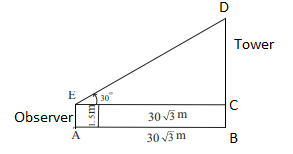AB = CE = 30√3 m

AE = BC = 1.5 m

In right triangle DEC,

tan 30 = CD/EC

1/√3 = CD/30√3

⇒ CD = 30√3/√3

⇒ CD = 30 m

Therefore, height of the tower = BD

= BC + CD

= 1.5 + 30

= 31.5 m

27. The total surface area of a solid right circular cylinder is 1540 cm2. If the height is four times the radius of the base, then find the height of the cylinder.

Solution:

Let r be the radius of the base and h be the height of the cylinder.

Given,

h = 4r

Total surface area = 1540 cm2

2πr(r + h) = 1540

2 × (22/7) × r(r + 4r) = 1540

r(5r) = (1540 × 7)/ (22 × 2)

5r2 = 35 × 7

r2 = 7 × 7

r = 7 m

Height of the cylinder = h = 4r = 4(7) = 28 cm

28. The smallest value of a collection of data is 12 and the range is 59. Find the largest value of the collection of data.

Solution:

Given,

Smallest number = 12

Range = 59

We know that,

Range = Largest number – Smallest number

59 = Largest number – 12

⇒ Largest number = 59 + 12 = 71

29. In tossing a fair coin twice, find the probability of getting:

(ii) Exactly one tail

Solution:

Sample space = S = {HH, HT, TH, TT}

n(S) = 4

(i) Let A be the event of getting two heads.

A = {HH}

Number of outcomes favourable to A = n(A) = 1

P(A) = n(A)/n(S)

= 1/4

(ii) Let B be the event of getting exactly one tail.

B = {HT, TH}

Number of outcomes favourable to B = n(B) = 2

P(B) = n(B)/n(S)

= 2/4

= 1/2

30. (a) If the volume of a solid sphere is 7241 1/7 cu.cm, then find its radius. (take π = 22/7)

Solution:

Let r be the radius of a solid sphere.

Given,

Volume of sphere = 7241 1/7 cu.cm

(4/3)πr3 = 50688/7

(4/3) × (22/7) × r3 = 50688/7

r3 = (50688 × 3)/ (4 × 22)

r3 = 1728

r3 = 43 × 33

⇒ r = 4 × 3

⇒ r = 12

Hence, the radius of the sphere = 12 cm

OR

(b) If x = a sec θ + b tan θ and y = a tan θ + b sec θ, then prove that: x2 – y2 = a2 – b2

Solution:

Given,

x = a sec θ + b tan θ

y = a tan θ + b sec θ

LHS = x2 – y2

= (a sec θ + b tan θ)2 – (a tan θ + b sec θ)2

= a2 sec2θ + b2 tan2θ + 2ab sec θ tan θ – a2 tan2θ – b2 sec2θ – 2ab tan θ sec θ

= a2(sec2θ – tan2θ) – b2(sec2θ – tan2θ)

= a2(1) – b2(1)

= a2 – b2

= RHS

Hence proved.

SECTION – III

31. Let A = {a, b, c, d, e, f, g, x, y, z}, B = {1, 2, c, d, e} and C = {d, e, f, g, 2, y}. Verify A\ (B ⋃ C) = (A\B) ⋂ (A\C).

Solution:

Given,

A = {a, b, c, d, e, f, g, x, y, z}, B = {1, 2, c, d, e} and C = {d, e, f, g, 2, y}

B ⋃ C = {1, 2, c, d, e} ⋃ {d, e, f, g, 2, y}

= {1, 2, c, d, e, f, g}

A\ (B ⋃ C) = {a, b, c, d, e, f, g, x, y, z} \ {1, 2, c, d, e, f, g}

= {a, b, x, z}….(i)

A\B = {a, b, c, d, e, f, g, x, y, z} \ {1, 2, c, d, e}

= {a, b, f, g, x, y, z}

A\C = {a, b, c, d, e, f, g, x, y, z} \ {d, e, f, g, 2, y}

= {a, b, c, x, z}

(A\B) ⋂ (A\C) = {a, b, f, g, x, y, z} ⋂ {a, b, c, x, z}

= {a, b, x, z}….(ii)

From (i) and (ii),

A\ (B ⋃ C) = (A\B) ⋂ (A\C)

Hence verified.

32. Let A = {6, 9, 15, 18, 21}, B = {1, 2, 4, 5, 6} and f : A → B be defined by f(x) = (x – 3)/3. Represent f by:

(i) an arrow diagram

(ii) a set of ordered pairs

(iii) a table

(iv) a graph

Solution:

Given,

f(x) = (x – 3)/3

f(6) = (6 – 3)/3 = 3/3 = 1

f(9) = (9 – 3)/3 = 6/3 = 2

f(15) = (15 – 3)/3 = 12/3 = 4

f(18) = (18 – 3)/3 = 15/3 = 5

f(21) = (21 – 3)/3 = 18/3 = 6

(i) An arrow diagram: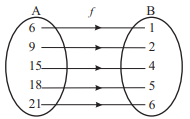(ii) The set of ordered pairs

f = {(6, 1), (9, 2), (15, 4), (18, 5), (21, 6)}

(iii) Table

 x 6 9 15 18 21 f(x) 1 2 4 5 6

(iv) Graph: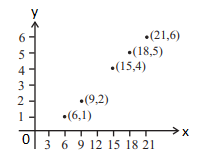33. Find the sum of the first 2n terms of the series 12 – 22 + 32 – 42 +….

Solution:

Given,

12 – 22 + 32 – 42 +….

= 1 – 4 + 9 – 16 + 25 – ….. to 2n terms

= (1 – 4) + (9 – 16) + (25 – 36) + …..to n terms (after grouping)

= -3 + (-7) + (-11) + ….. n terms

This is an AP with a = -3, d = -4

Hence, the required sum = n/2 [2a + (n – 1)d]

= n/2 [2(-3) + (n – 1)(-4)]

= (n/2) [-6 – 4n + 4]

= (n/2) [-4n – 2]

= (-2n/2) (2n + 1)

= -n(2n + 1)

34. Find the sum of the first n terms of the series 7 + 77 + 777 +…

Solution:

Given,

7 + 77 + 777 +…

Sum of first n terms

Sn = 7 + 77 + 777 + ….. + (n terms)

Sn = 7(1 + 11 + 111 + …. + n terms)

= (7/9) [9 + 99 + 999 + … + n terms]

= (7/9) [(10 – 1) + (100 – 1) + (1000 – 1) + …. + (10n – 1)]

= (7/9) [(10 + 102 + 103 + … + 10n) – (1 + 1 + 1 + … + n terms)]

= (7/9) {[10(10n – 1)/ (10 – 1)] – n}

= (7/9) {[10(10n – 1)/9] – n}

= (70/81) (10n – 1) – 7n/9

35. The speed of a boat in still water is 15 km/hr. It goes 30 km upstream and returns downstream to the original point in 4 hrs.30minutes. Find the speed of the stream.

Solution:

Given,

Speed in still water = 15 km/hr

Total time taken = 4 hrs 30 min = 4 1/2 hr = 9/2 hrs

Let x km/hr be the speed of the stream.

Speed of the boat during upstream = 15 – x

Speed of the boat during downstream = 15 + x

Time taken for upstream = T1 = 30/(15 – x)

Time taken for downstream = T2 = 30/(15 + x)

According to the given,

T1 + T2 = 9/2

[30/ (15 – x)] + [30/ (15 + x)] = 9/2

30 [(15 + x + 15- x)/ (15 – x)(15 + x)] = 9/2

30/(225 – x2) = (9/ 2 × 30)

30/(225 – x2) = 3/20

⇒ 600 = 675 – 3x2

⇒ 3x2 = 675 – 600

⇒ x2 = 75/3

⇒ x2 = 25

⇒ x = 5

Therefore, speed of the stream = 5 km/hr

36. Find the value of a and b if 16x4 – 24x3 + (a – 1)x2 + (b + 1)x + 49 is a perfect square.

Solution: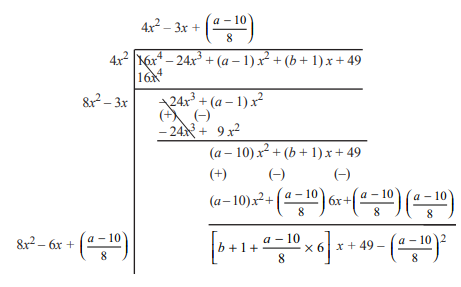Given that, the polynomial is a perfect square.

Therefore, remainder = 0

b + 1 + [(a – 10)/8] × 6 = 0 ….(i)

49 – [(a – 10)/8]2 = 0

⇒ [(a – 10)/8]2 = 49

⇒ (a – 10)/8 = 7

⇒ a = 10 = 56

⇒ a = 56 + 10 = 66

Substituting a = 66 in (i),

b + 1 + [(66 – 10)/8] × 6 = 0

b + 1 + (56/8) × 6 = 0

b + 1 + 42 = 0

b = -43

Therefore, a = 66 and b = -43.

37.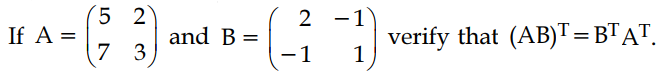Solution:

Given,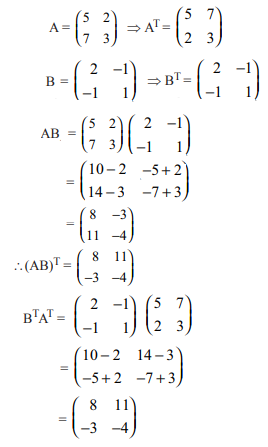Hence, (AB)T = BTAT

38. Find the area of the quadrilateral formed by the points (-4, -2), (-3, -5), (3, -2) and (2, 3).

Solution:

Given, vertices of the quadrilateral are (-4, -2), (-3, -5), (3, -2) and (2, 3).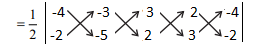= (1/2) [(20 + 6 + 9 – 4) – (6 – 15 – 4 – 12)]

= (1/2) [31 + 25]

= (1/2) × 56

= 28 sq.units

39. State and prove Pythagoras theorem.

Solution:

Pythagoras theorem – statement:

In a right angled triangle, the square of the hypotenuse is equal to the sum of the squares of the other two sides.

Given:

In a right triangle ABC, ∠B = 90°

To prove:

AC2 = AB2 + BC2

Construction:

Draw a perpendicular BD onto the side AC.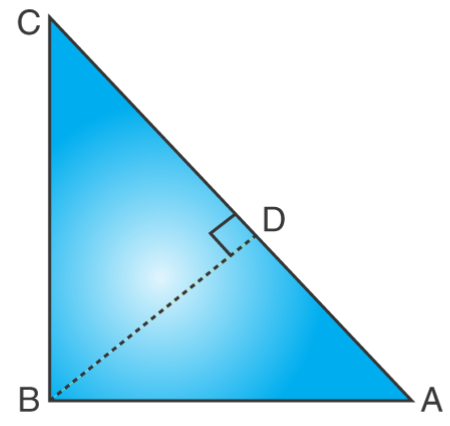We know that,

Therefore, AD/AB = AB/AC (by similarity)

AB2 = AD × AC …..(i)

Also, △BDC ~△ABC

Therefore, CD/BC = BC/AC (by similarity)

BC2 = CD × AC ….(ii)

AB2 + BC2 = AD × AC + CD × AC

AB2 + BC2 = AC (AD + CD)

Since, AD + CD = AC

Therefore, AC2 = AB2 + BC2

Hence, the Pythagorean theorem is proved.

40. A flag post stands on the top of a building. From a point on the ground, the angles of elevation of the top and bottom of the flag post are 60° and 45° respectively. If the height of the flag post is 10 m, find the height of the building. (√3 = 1.732)

Solution:

Let AB be the flag post and BC be the building.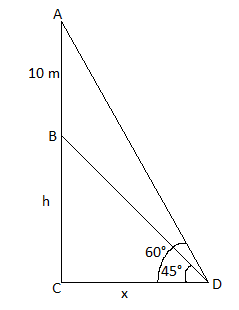In right triangle BCD,

tan 45° = BC/CD

1 = h/x

⇒ h = x ….(i)

In right triangle ACD,

tan 60° = AC/CD

√3 = (10 + h)/x

√3x = 10 + h

√3h = 10 + h [From (i)]

(√3 – 1)h = 10

h = 10/(√3 – 1)

h = [10/(√3 – 1)] [(√3 + )/ (√3 + 1)]

h = 10(√3 + 1)/ (3 – 1)

h = 10(1.732 + 1)/2

= 5 (2.732)

= 13.66 m

Therefore, the height of the building is 13.66 m.

41. The perimeter of the ends of a frustum of a cone are 44 cm and 8.4π cm. If the depth is 14 cm, then find its volume.

Solution:

Given,

Depth of the frustum of a cone = h = 14 cm

Perimeter of circular base with radius R = 44 cm

2πR = 44

(22/7) R = 22

R = 7 cm

Perimeter of circular end with radius r = 8.4π cm

2πr = 8.4π

r = 8.4/2

r = 4.2 cm

Volume of the frustum = (1/3)πh[R2 + r2 + Rr]

= (1/3) × (22/7) × 14 × [72 + 4.22 + (7)(4.2)]

= (44/3) × [49 + 17.64 + 29.4]

= (44/3) × 96.04

= 1408.58 cm3

42. The length, breadth and height of a solid metallic cuboid are 44 cm, 21 cm and 12 cm respectively. It is melted and a solid cone is made out of it. If the height of the cone is 24 cm, then find the diameter of its base.

Solution:

Given, dimensions of the cuboid are:

Length = l = 44 cm

Breadth = b = 21 cm

Height = h = 12 cm

Let r be the radius of the cone.

Height of the cone = H = 24 cm (given)

Volume of cone = Volume of cuboid

(1/3)πr2H = lbh

(1/3) × (22/7) × r2 × 24 = 44 × 21 × 21

r2 = (44 × 21 × 12 × 7 × 3)/ (22 × 24)

r2 = 7 × 3 × 7 × 3

r2 = (7 × 3)2

r = 21 cm

Diameter of the base of cone = 2r = 2(21) = 42 cm

43. Find the coefficient of variation of the following data.

18, 20, 15, 12, 25

Solution:

Given,

18, 20, 15, 12, 25

Mean = (18 + 20 + 15 + 12 + 25)/5

= 90/5

= 18

 x d = x – 18 d2 18 0 0 20 2 4 15 -3 9 12 -6 36 25 7 49 ∑d = 0 ∑d2 = 98

Standard deviation (σ) = √(∑d2/n)

= √(98/5)

= √19.6

= 4.428

Coefficient of variation = (standard deviation/mean) × 100

= (4.428/18) × 100

= 442.8/18

= 24.6

44. If a die is rolled twice, find the probability of getting an even number in the first time or a total of 8.

Solution:

Total number of outcomes = n(S) = 62 = 36

Let A be the event of getting an even number for the first time.

A = {(2, 1), (2, 2), (2, 3) (2, 4), (2, 5), (2, 6), (4, 1), (4, 2), (4, 3), (4, 4), (4, 5), (4, 6), (6, 1), (6, 2), (6, 3), (6, 4), (6, 5), (6, 6)}

n(A) = 18

P(A) = n(A)/n(S) = 18/36

Let B be the event of getting a sum of numbers on dice 8.

B = {(2, 6), (6, 2), (3, 5), (5, 3), (4, 4)}

n(B) = 5

P(B) = n(B)/n(S) = 5/36

A ⋂ B = {(2, 6), (6, 2), (4, 4)}

n(A ⋂ B) = 3

P(A ⋂ B) = n(A ⋂ B)/n(S) = 3/36

P(A ⋃ B) = P(A) + P(B) – P(A ⋂ B)

= (18/36) + (5/36) – (3/36)

= (18 + 5 – 3)/36

= 20/36

= 5/9

Hence, the required probability is 5/9.

45. (a) Find the GCD of the following polynomials 3x4 + 6x3 – 12x2 – 24x and 4x4 + 14x3 + 8x2 – 8x.

Solution:

Let f(x) = 3x4 + 6x3 – 12x2 – 24x

= 3x(x3 + 2×2 – 4x – 8)

g(x) = 4x4 + 14x3 + 8x2 – 8x

= 2x(2x3 + 7x2 + 4x – 4)

Now, let us find the GCD of the below polynomials.

x3 + 2x2 – 4x – 8 and 2x3 + 7x2 + 4x – 4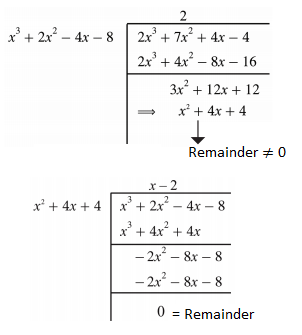Thus, the common factor of x3 + 2x2 – 4x – 8 and 2x3 + 7x2 + 4x – 4 is x2 + 4x + 4.

Also, the common factor of 3x and 2x is x.

Therefore, the required GCD the given polynomials = x(x2 + 4x + 4).

OR

(b) A straight line cuts the coordinate axes at A and B. If the midpoint of AB is (3, 2), then find the equation of AB.

Solution:

Let A(a, 0) and B(0, b) the points on the x-axis and y-axis respectively.

Given that, (3, 2) is the midpoint of AB.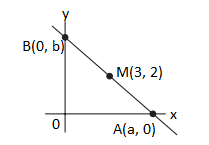M(3, 2) = Midpoint of AB

(3, 2) = [(a + 0)/2, (0 + b)/2]

(3, 2) = (a/2, b/2)

⇒ a = 6 and b = 4

The equation of line passing through A and B is (x/a) + (y/b) = 1

⇒ (x/6) + (y/4) = 1

⇒ (2x + 3y)/12 = 1

⇒ 2x + 3y = 12

Hence, the required equation is 2x + 3y – 12 = 0

SECTION – IV

46. (a) Draw the two tangents from a point which is 10 cm away from the centre of a circle of radius 6 cm. Also, measure the lengths of the tangents.

Solution: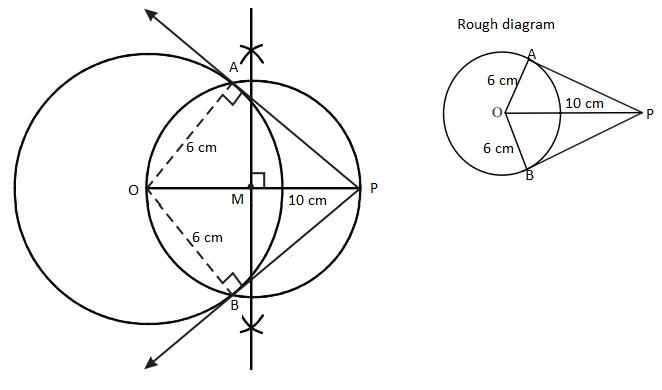Measure of length of the tangents = PA = PB = 8 cm

OR

(b) Construct a cyclic quadrilateral ABCD, given AB = 6 cm, ∠ABC = 70°, BC = 5 cm and ∠ACD = 30°.

Solution: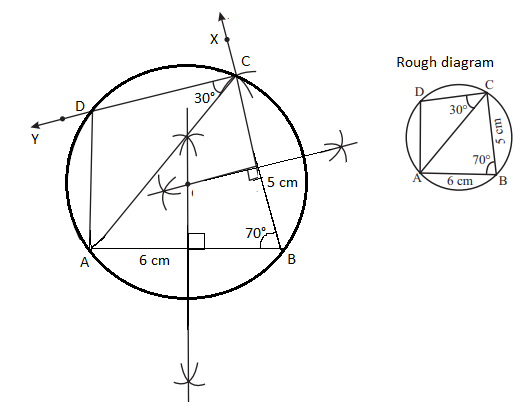47. (a) Solve graphically 2x2 + x – 6 = 0

Solution:

Given equation is:

2x2 + x – 6 = 0

Let y = 2x2 + x – 6

Plot the ordered pair of points (–3, 9), (–2, 0), (–1, –5), (0, –6), (1, –3), (2, 4) and (3, 15) on the graph.

 x -3 -2 -1 0 1 2 3 x2 9 4 1 0 1 4 9 2×2 18 8 2 0 2 8 18 x -3 -2 -1 0 1 2 3 -6 -6 -6 -6 -6 -6 -6 -6 y 9 0 -5 -6 -3 4 15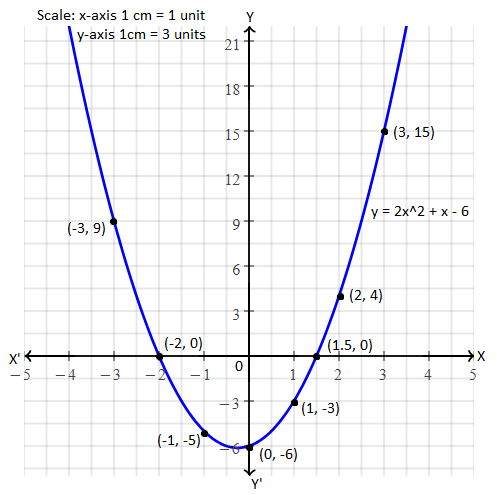The curve in the graph, cuts the x-axis at the points (–2, 0) and (1.5, 0).

Hence, the solution set is x = -2 and x = 1.5.

OR

(b) Draw the graph of xy = 20, x, y > 0. Use the graph to find y when x = 5, and to find x when y = 10.

Solution:

Given, xy = 20

 x 1 2 4 5 y 20 10 5 4

From the above table, it is clear that as x increases y decreases. This type of variation is called indirect variation.

y α 1/x

or

xy = k, where k = proportionality constant

Now,

1 × 20 = 2 × 10 = 4 × 5 = 20 = k

Thus, k = 20

Plot the points (1, 20), (2, 10), (4, 5) and (5, 4) in the graph.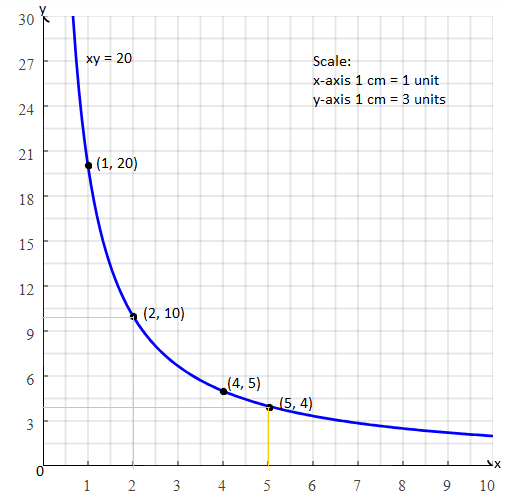When x = 5, y = 4

When y = 10, x = 2